# ML Aggarwal Solutions for Class 8 Maths Chapter 12 Linear Equations and Inequalities in One Variable

ML Aggarwal Solutions for Class 8 Maths Chapter 12 Linear Equations and Inequalities in One Variable has step by step answers to help students in exam preparation. These solutions are the best study material in clearing students’ doubts on the spot. Practising ML Aggarwal solutions regularly helps students to understand the concepts effortlessly. For more conceptual knowledge, students can make use of ML Aggarwal Solutions for Class 8 Maths Chapter 12 Linear Equations and Inequalities in One Variable PDF, from the links provided here.

Chapter 12 explains the problems based on linear equations and Inequalities in One Variable with examples. The solutions are prepared by expert teachers, with the intention of helping students, to improve their skills in Mathematics. The solutions are available online and offline, as per the students needs.

## Download the PDF of ML Aggarwal Solutions for Class 8 Maths Chapter 12 Linear Equations and Inequalities in One Variable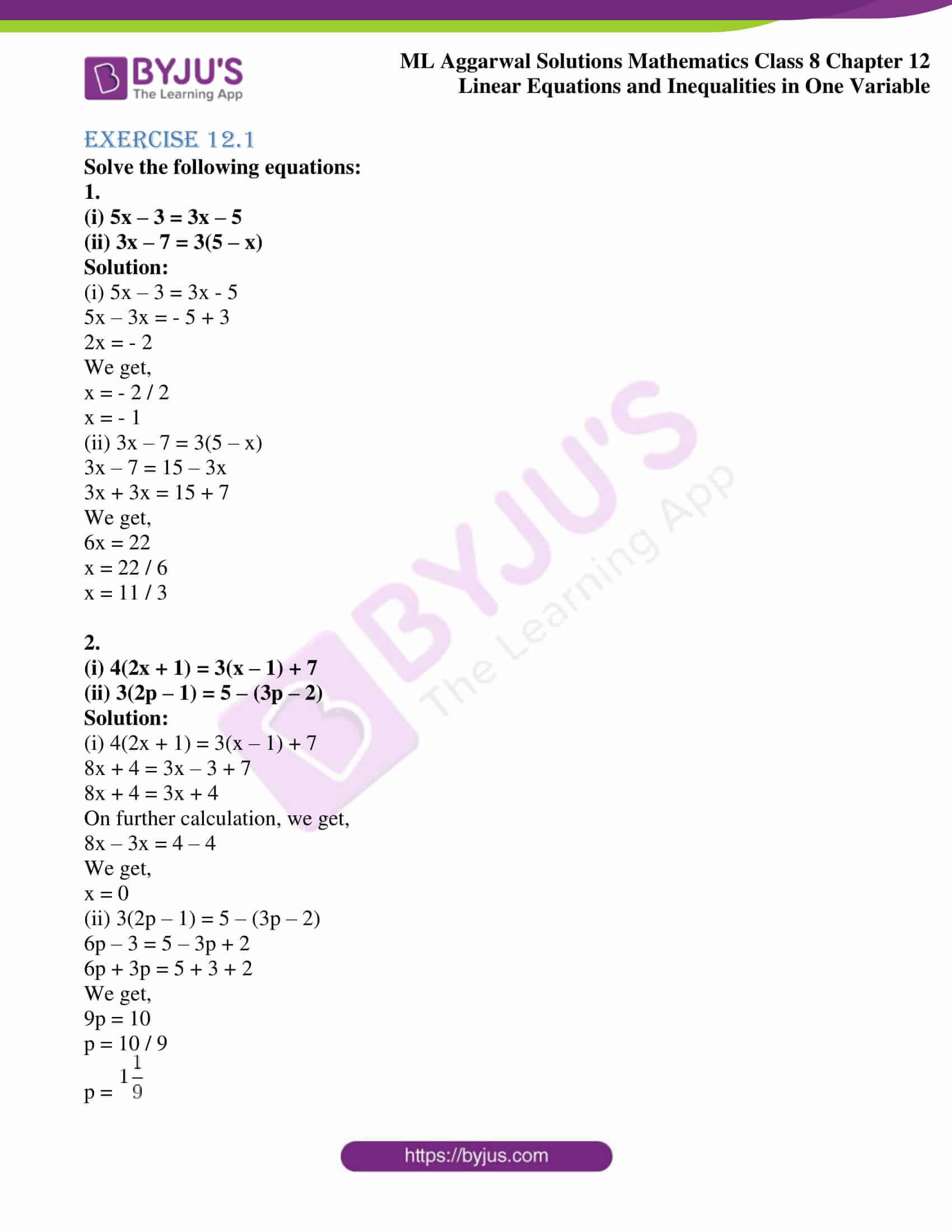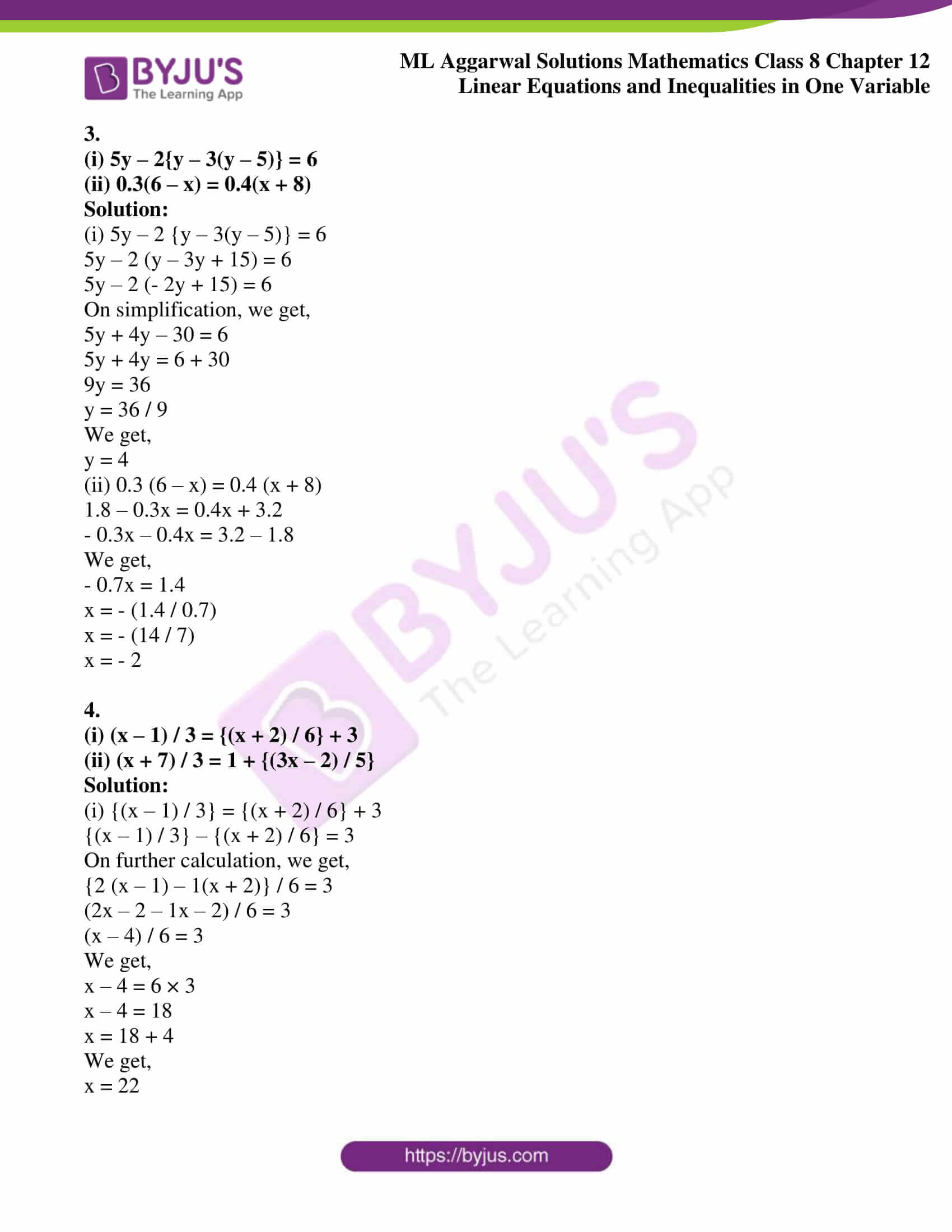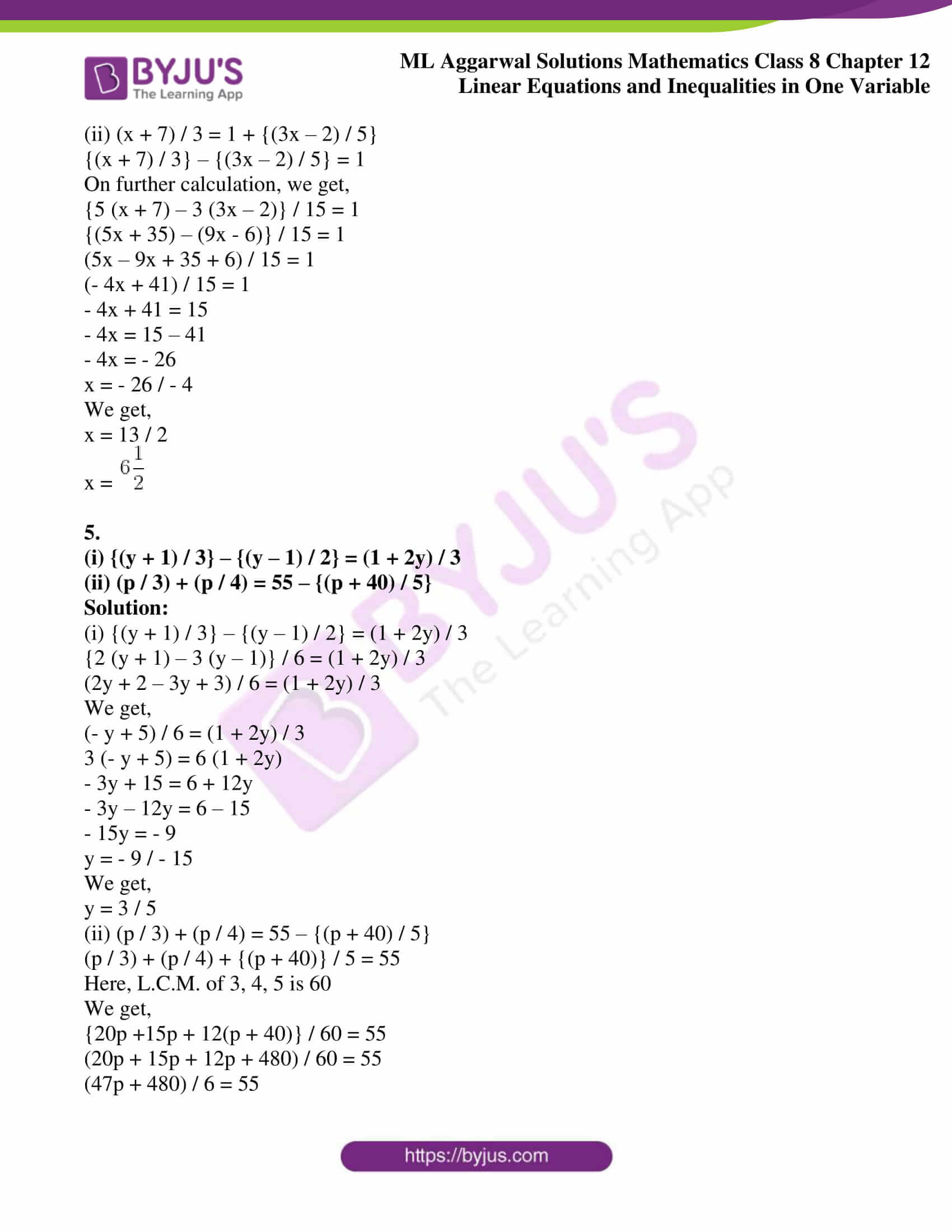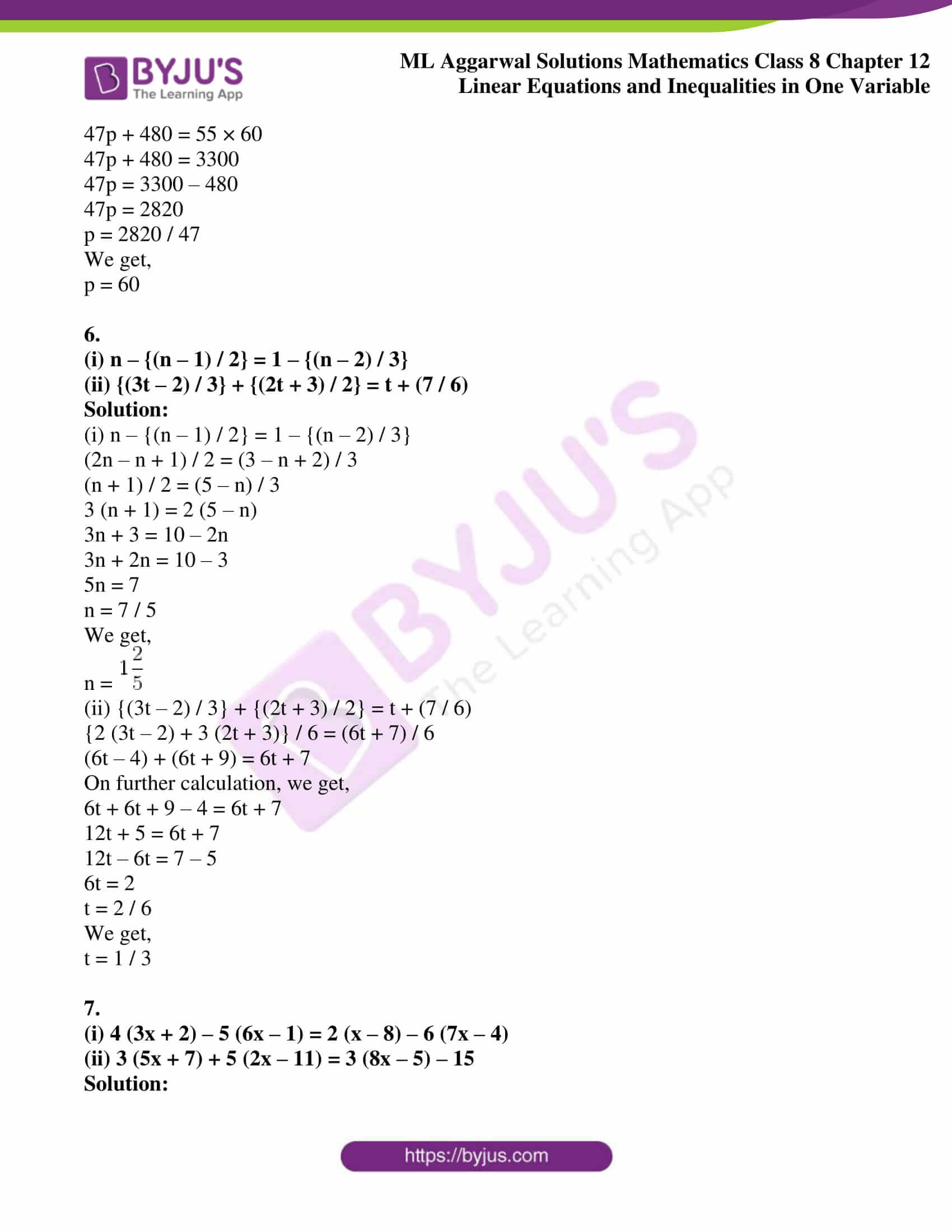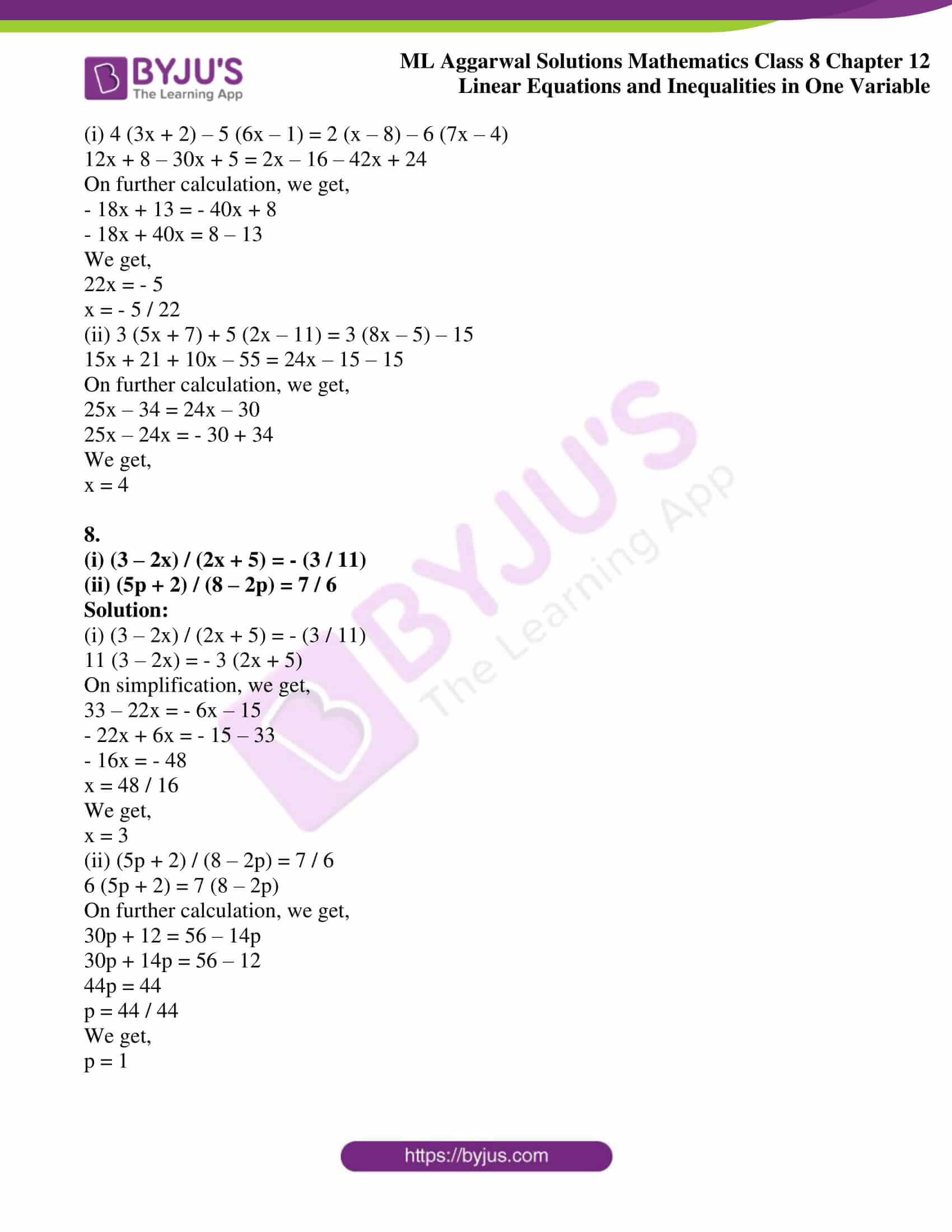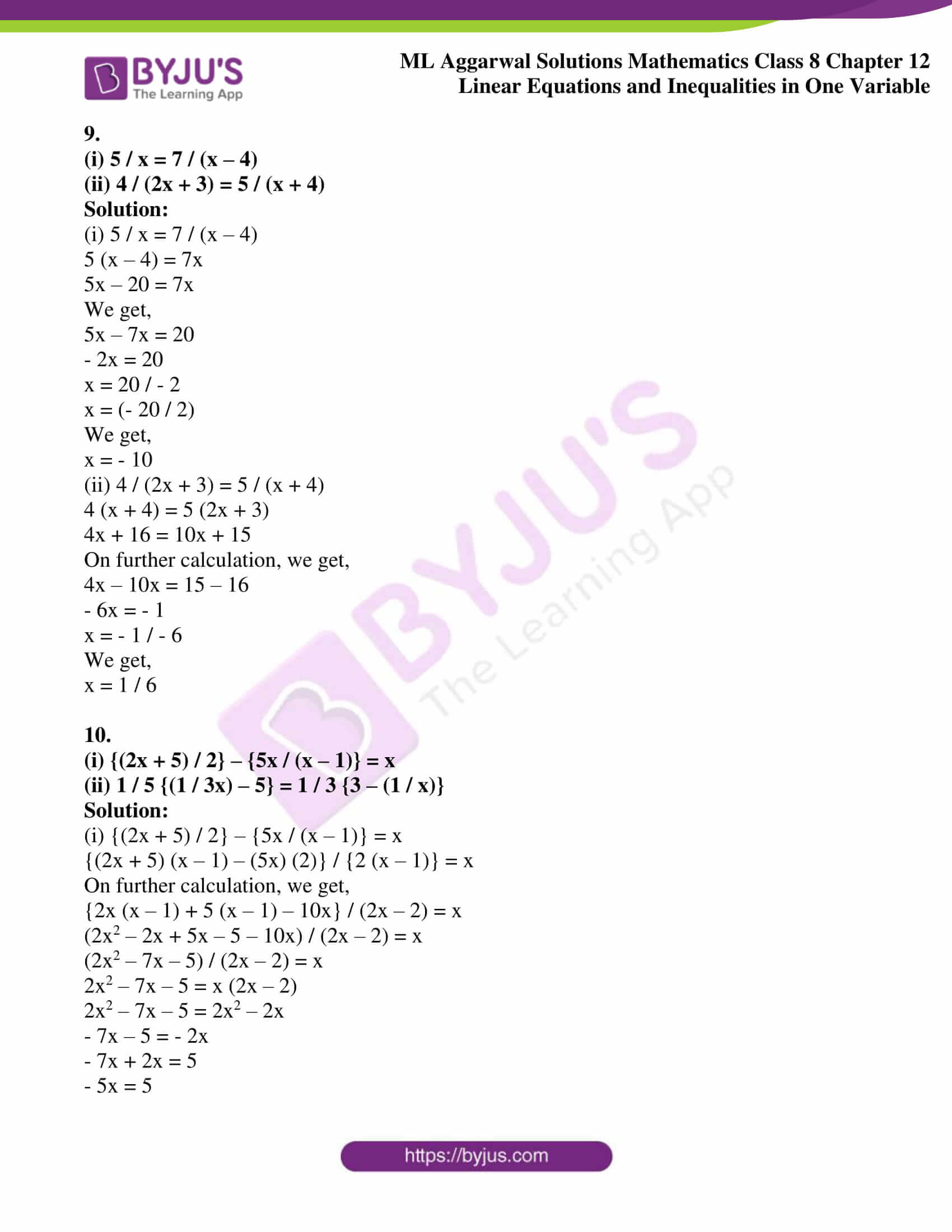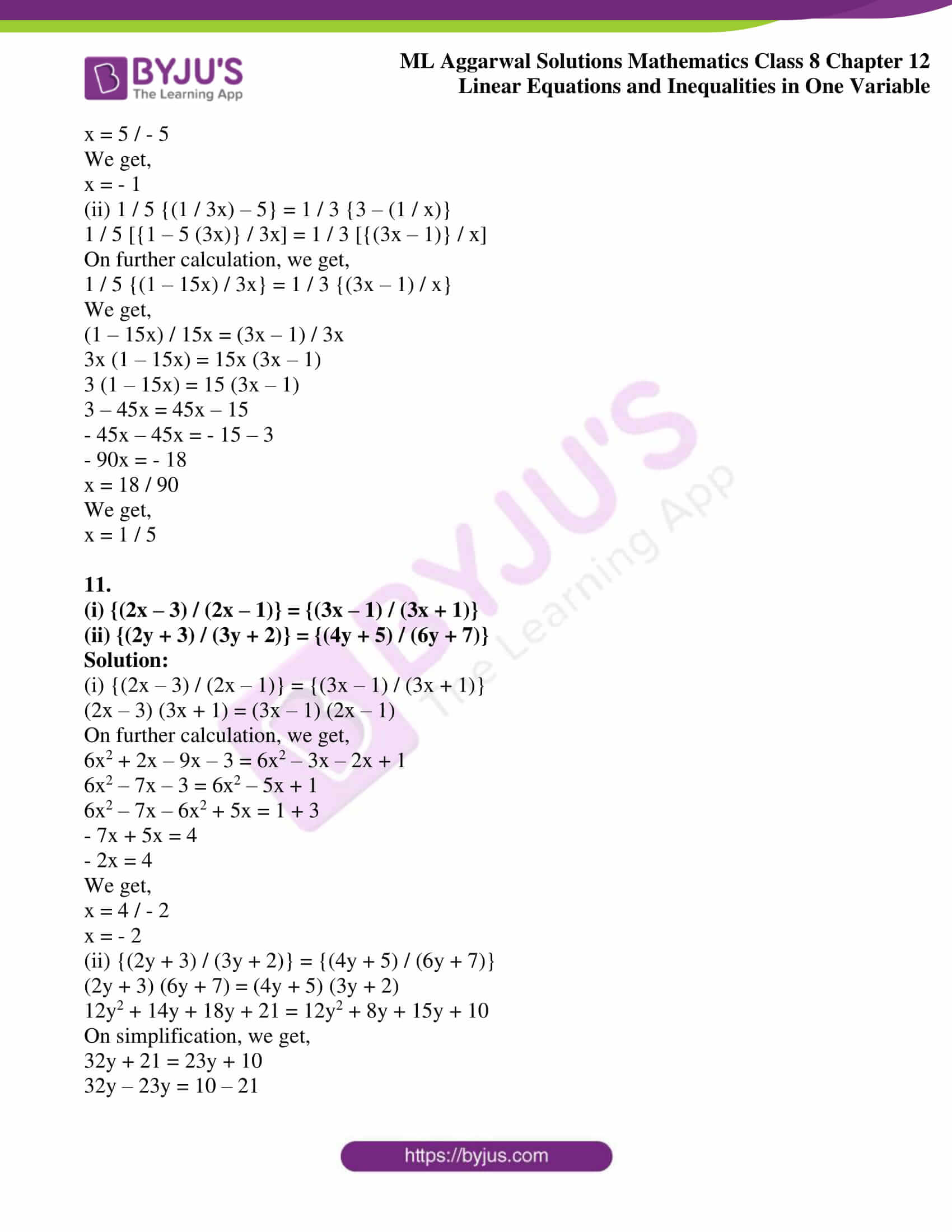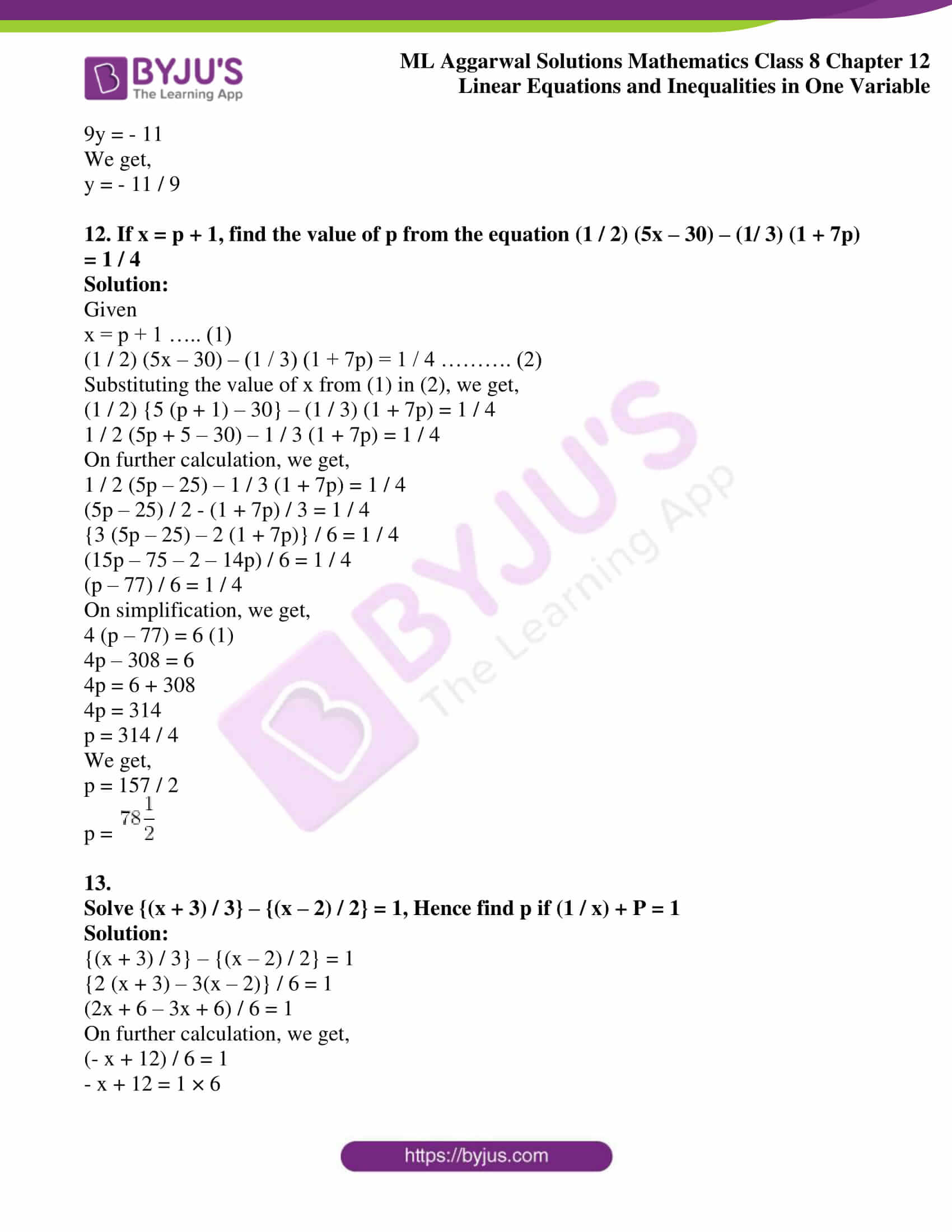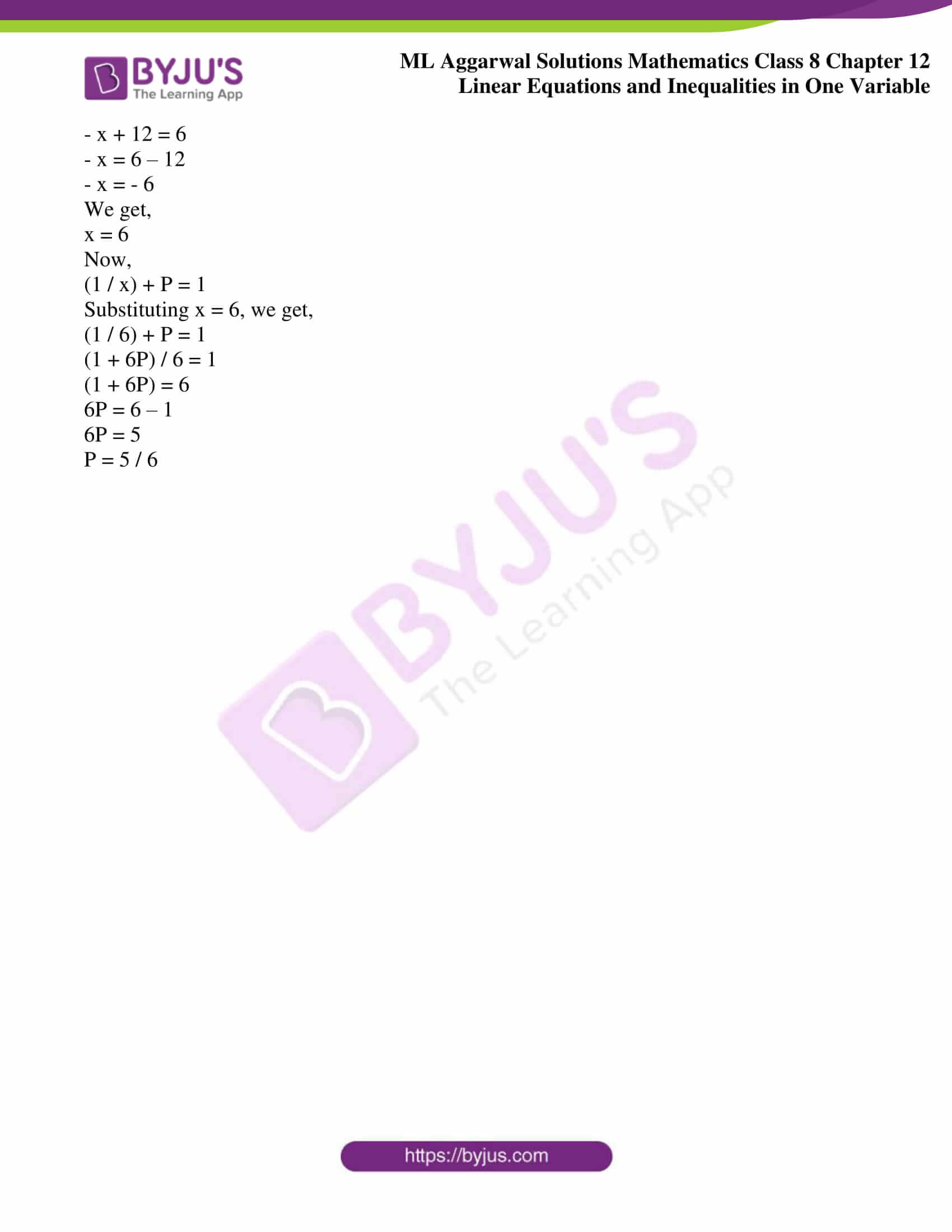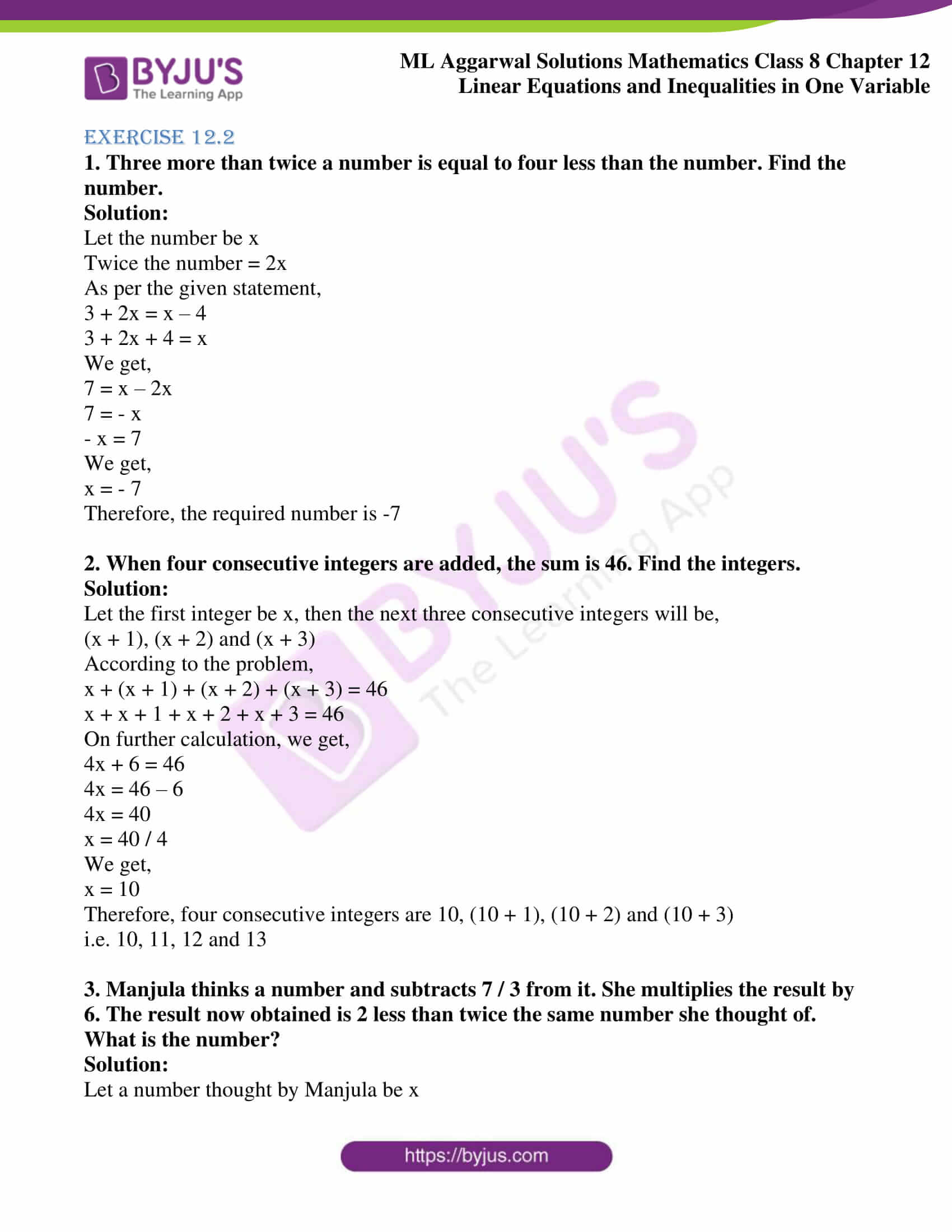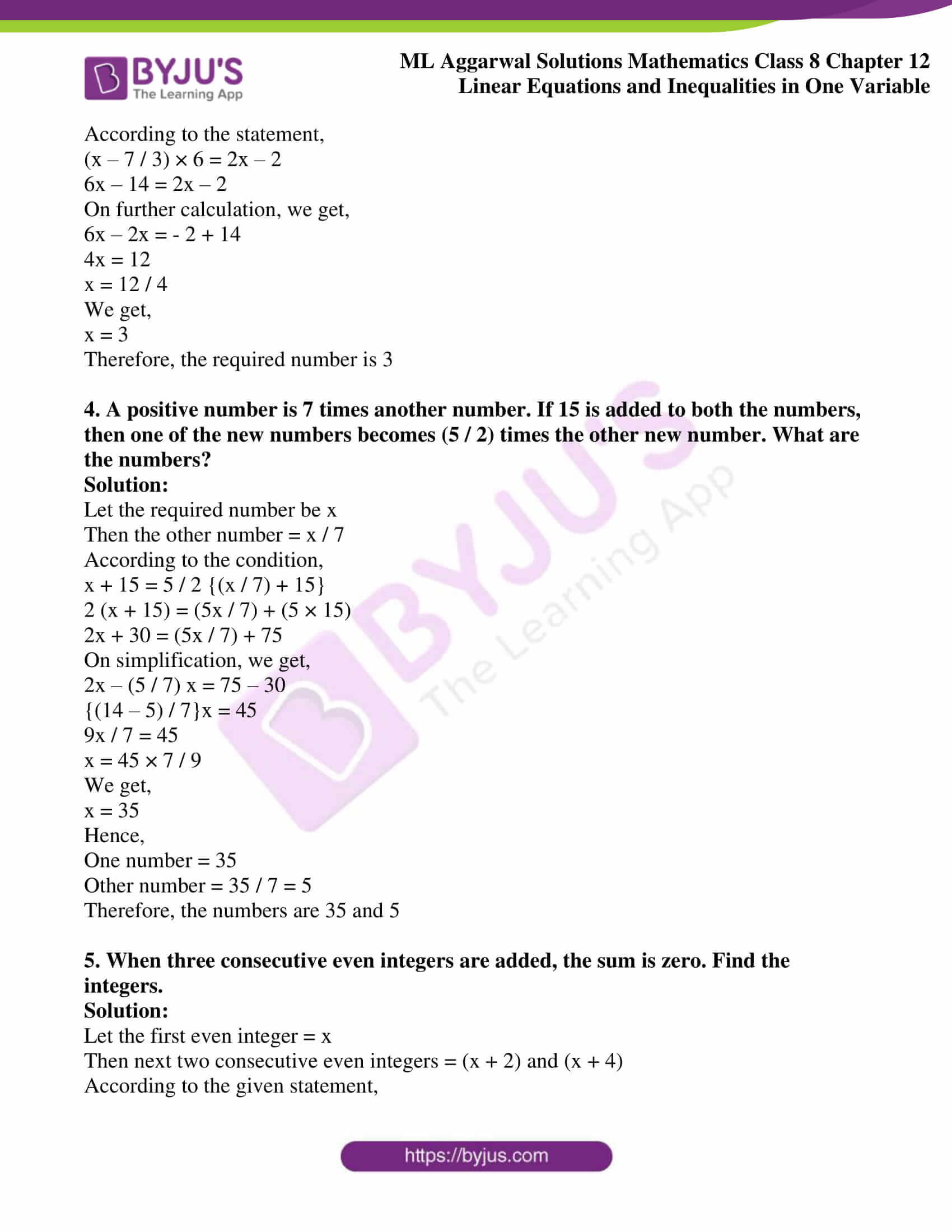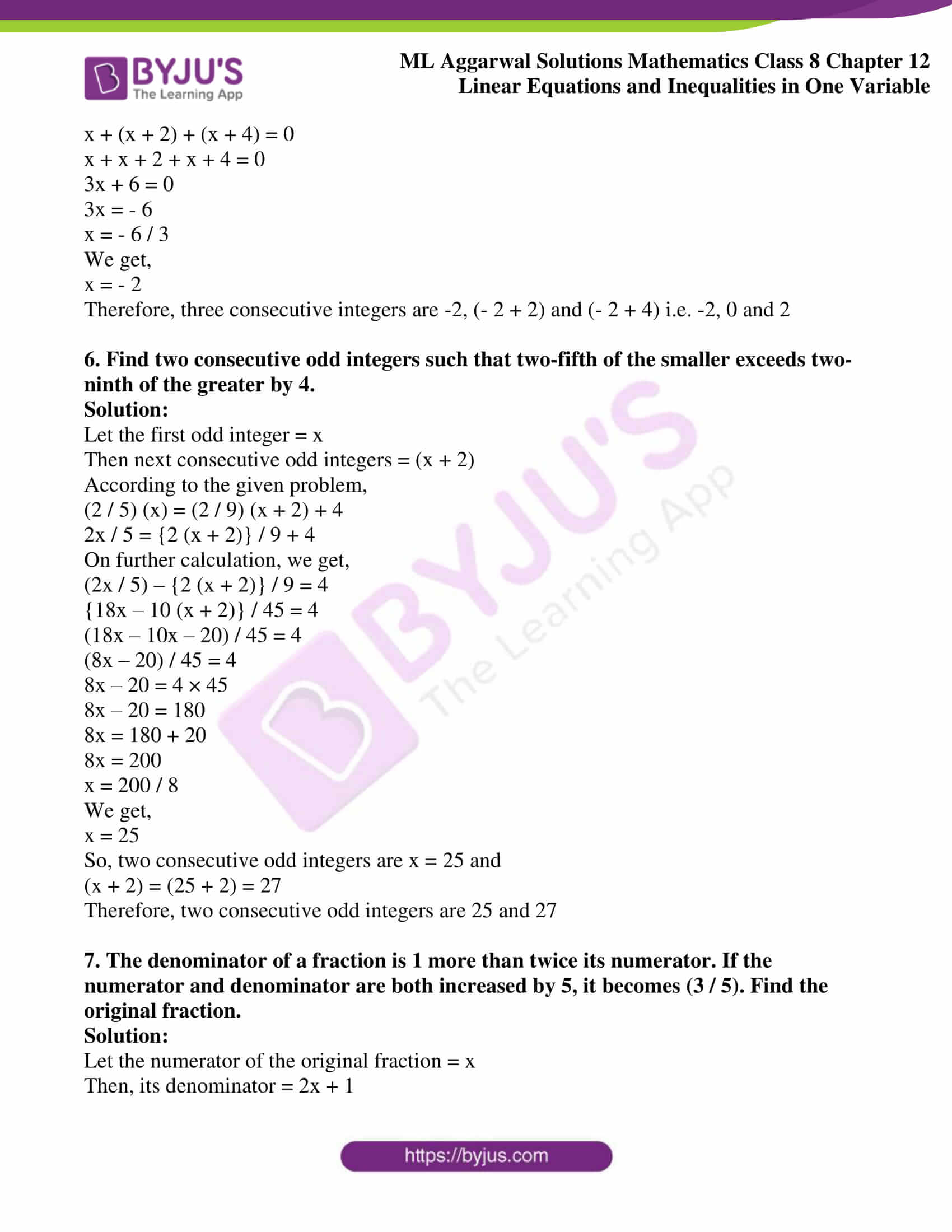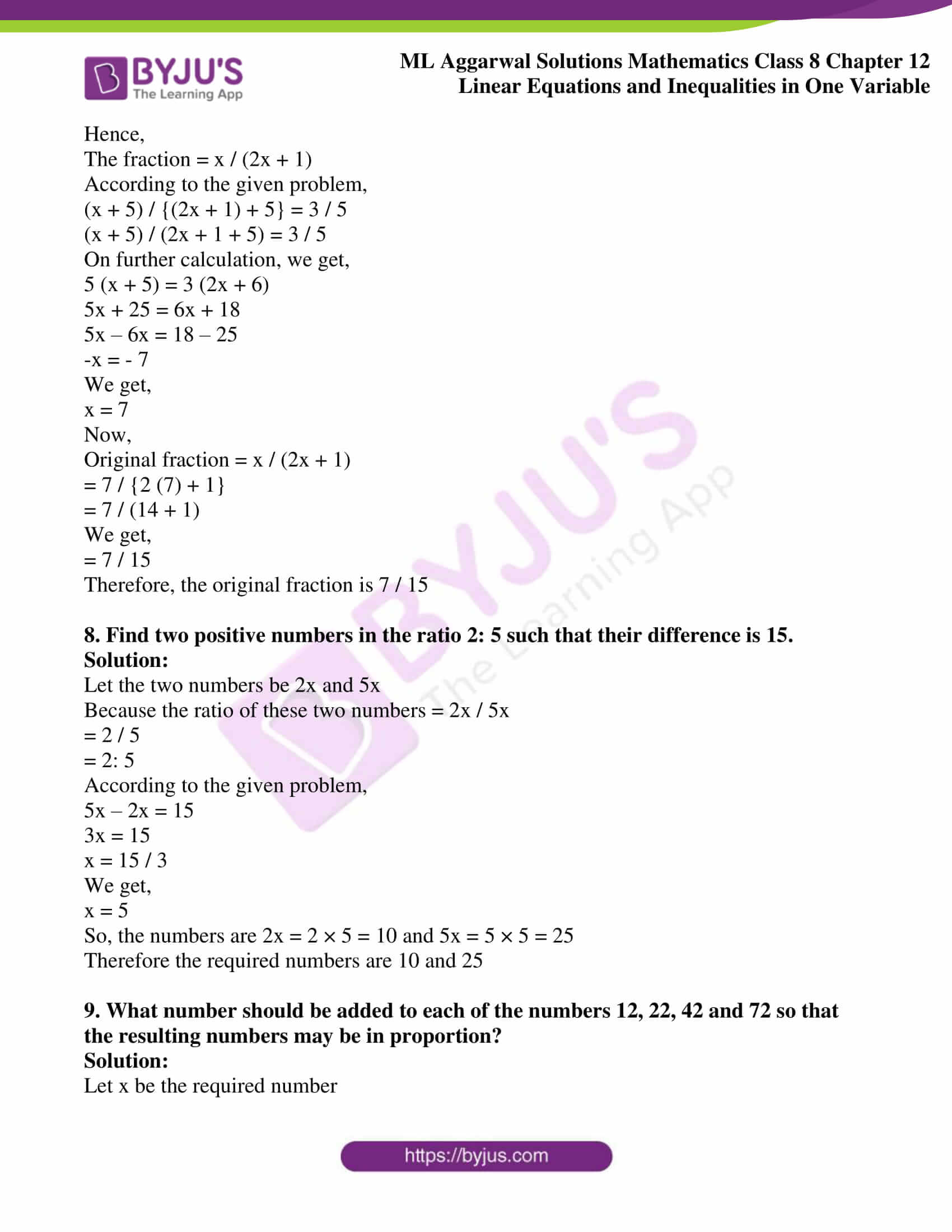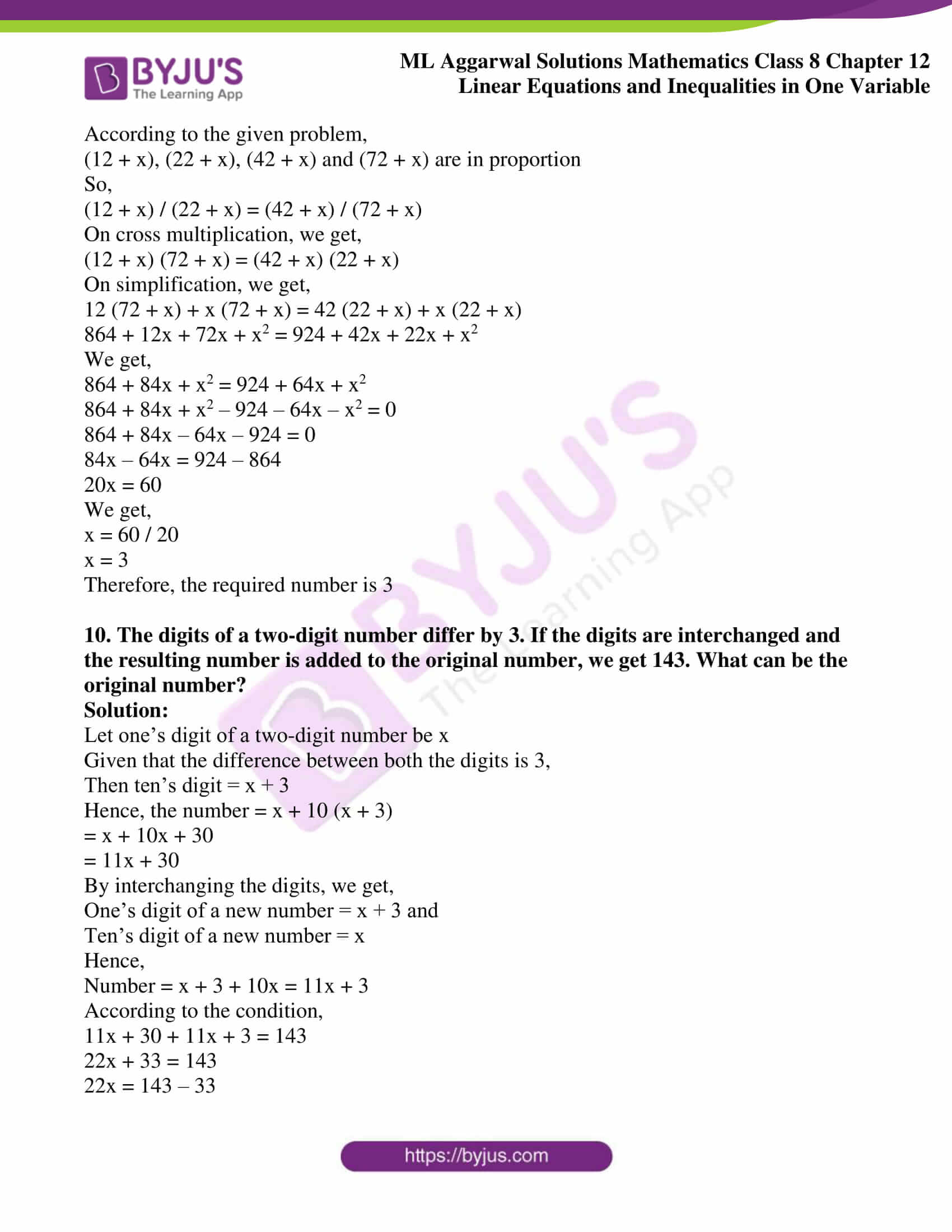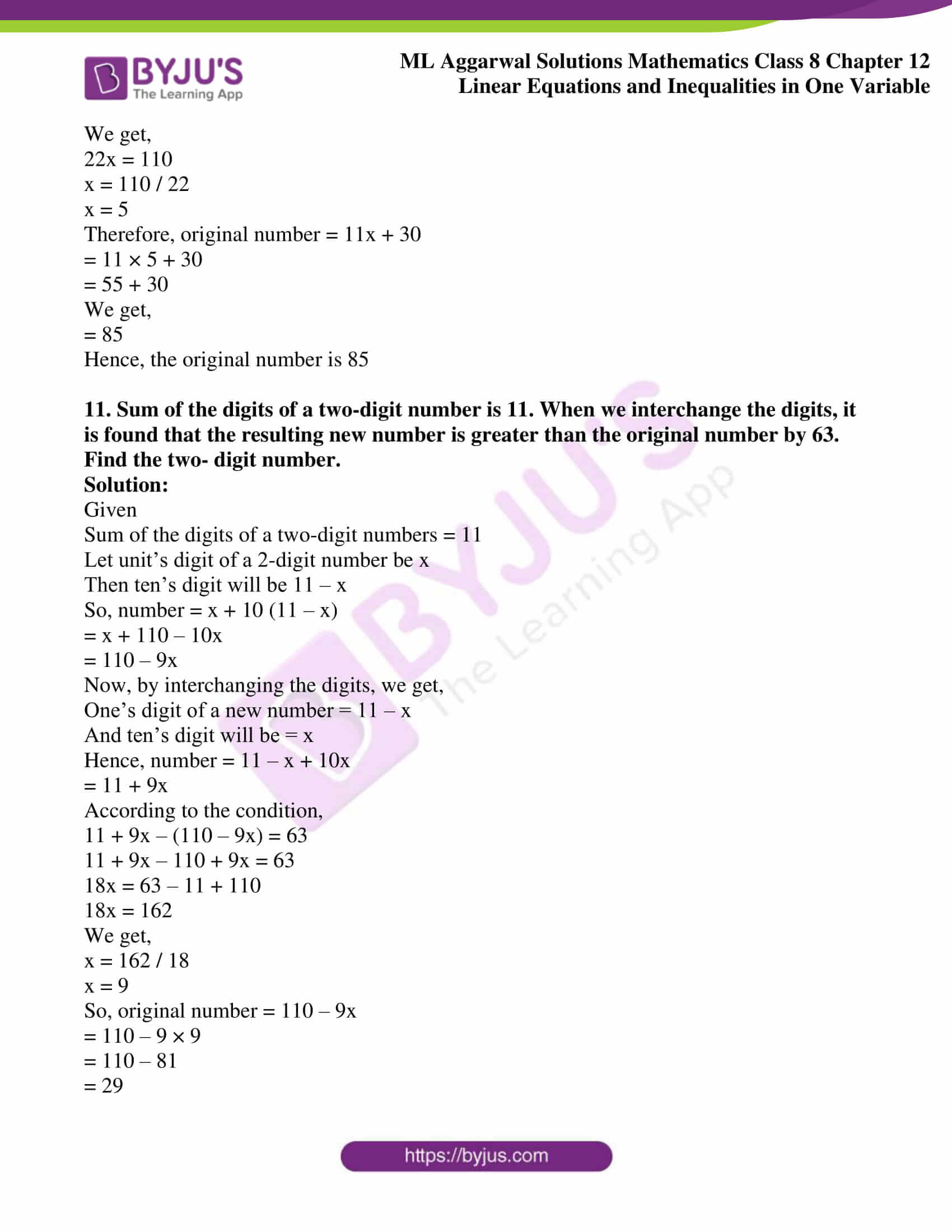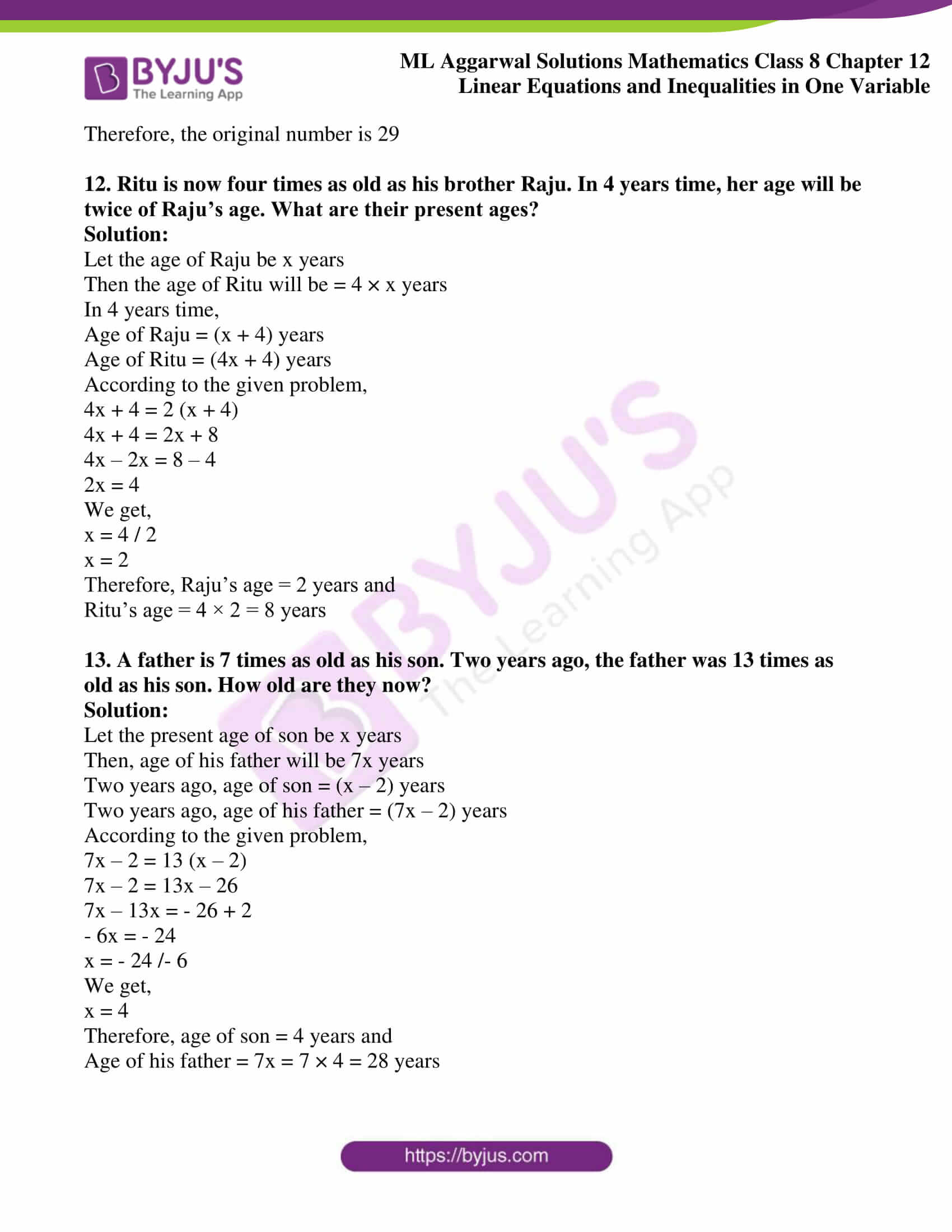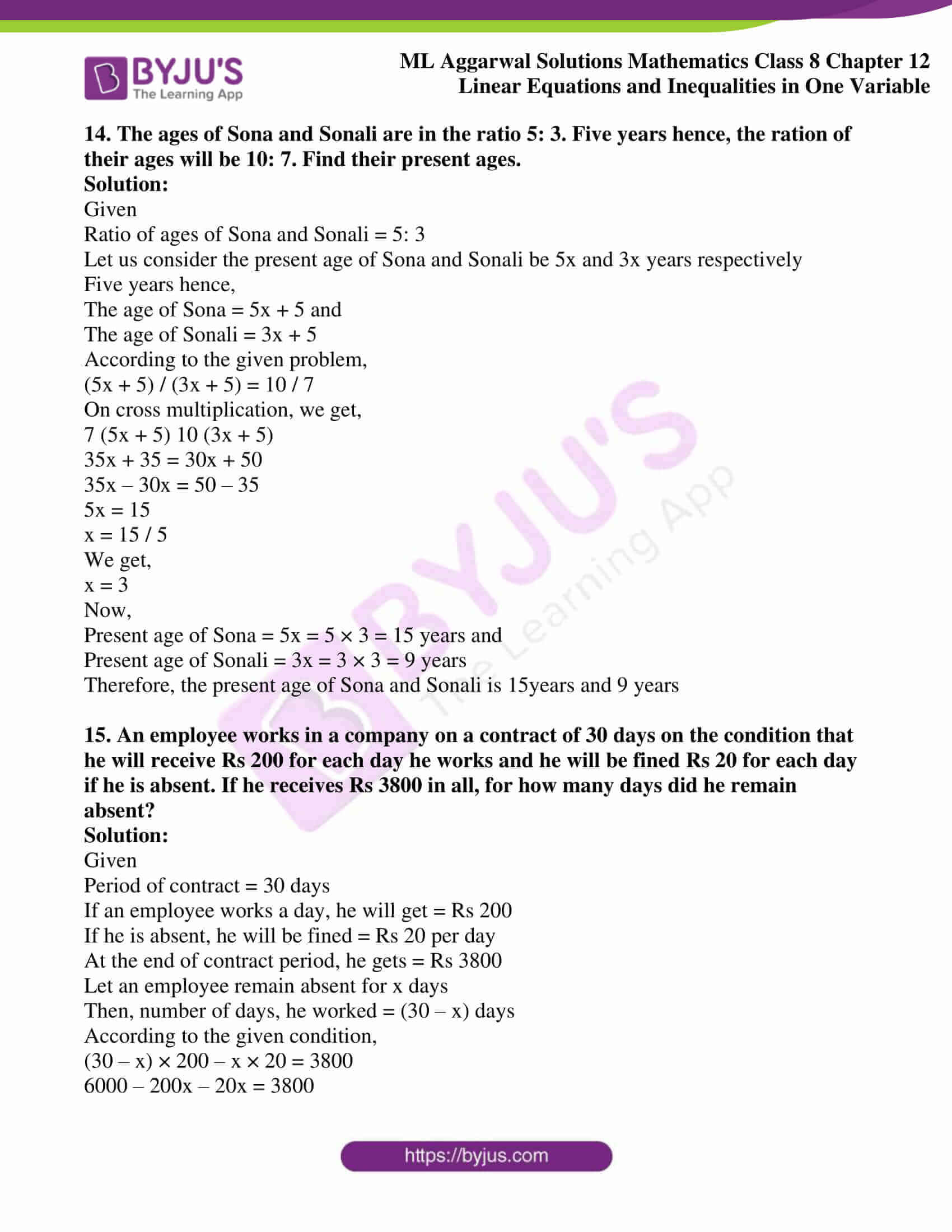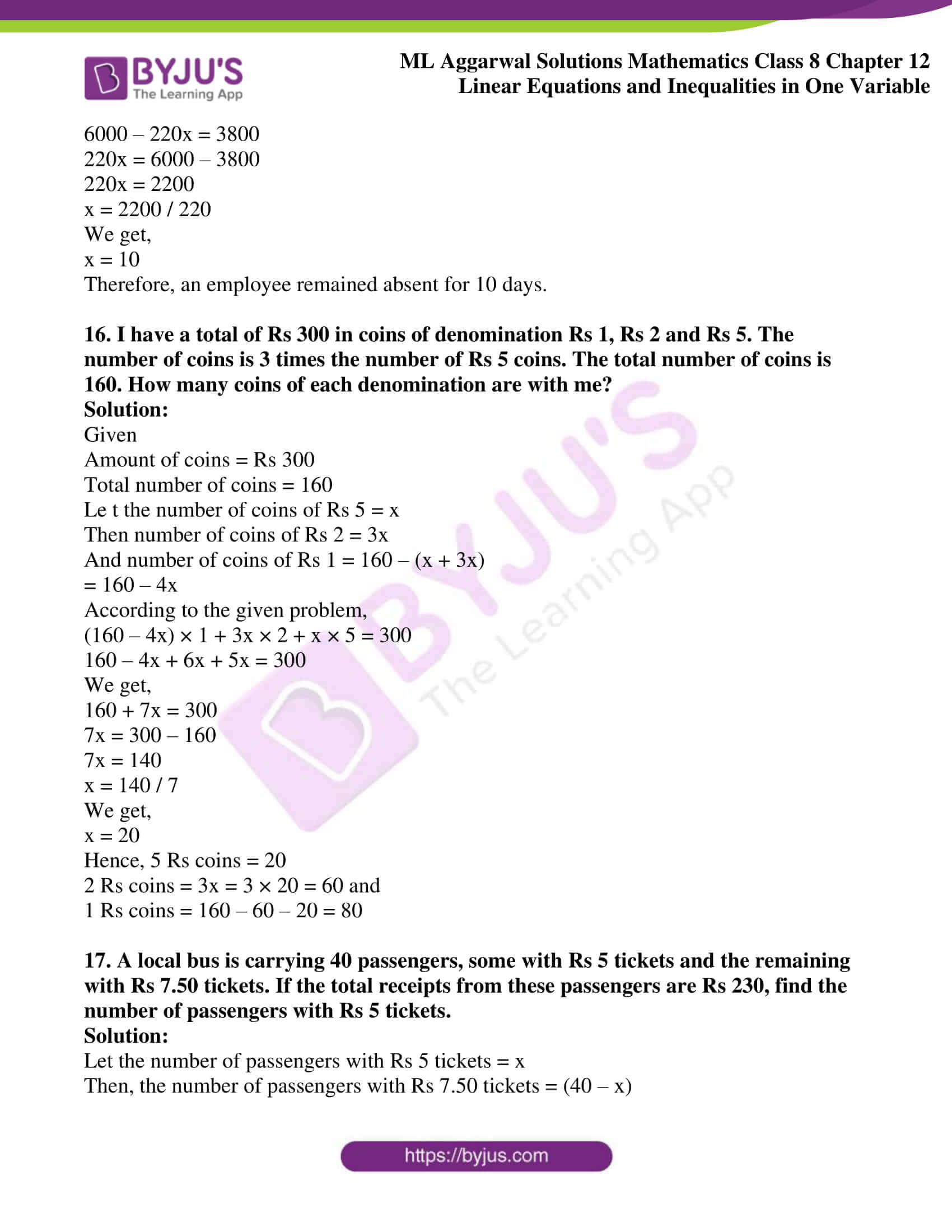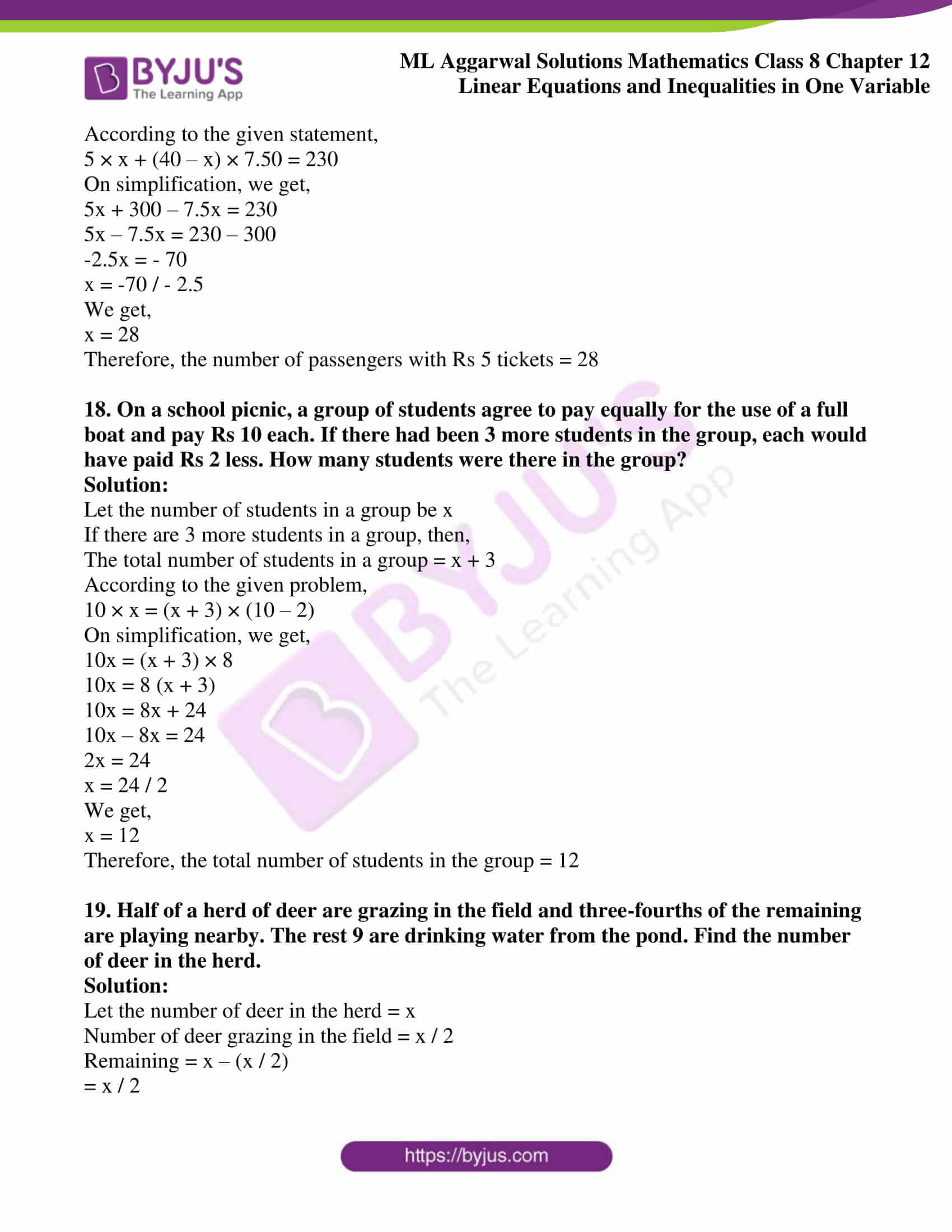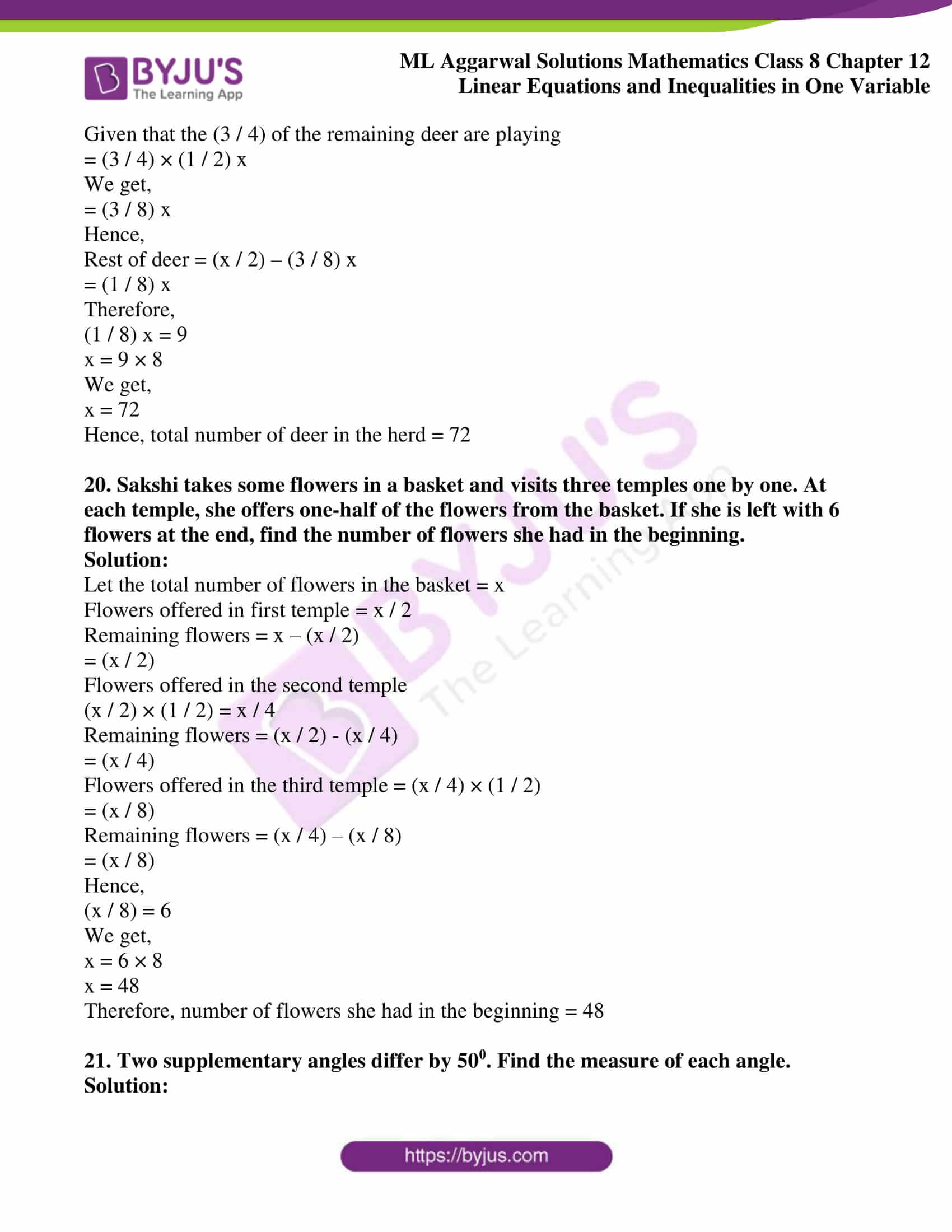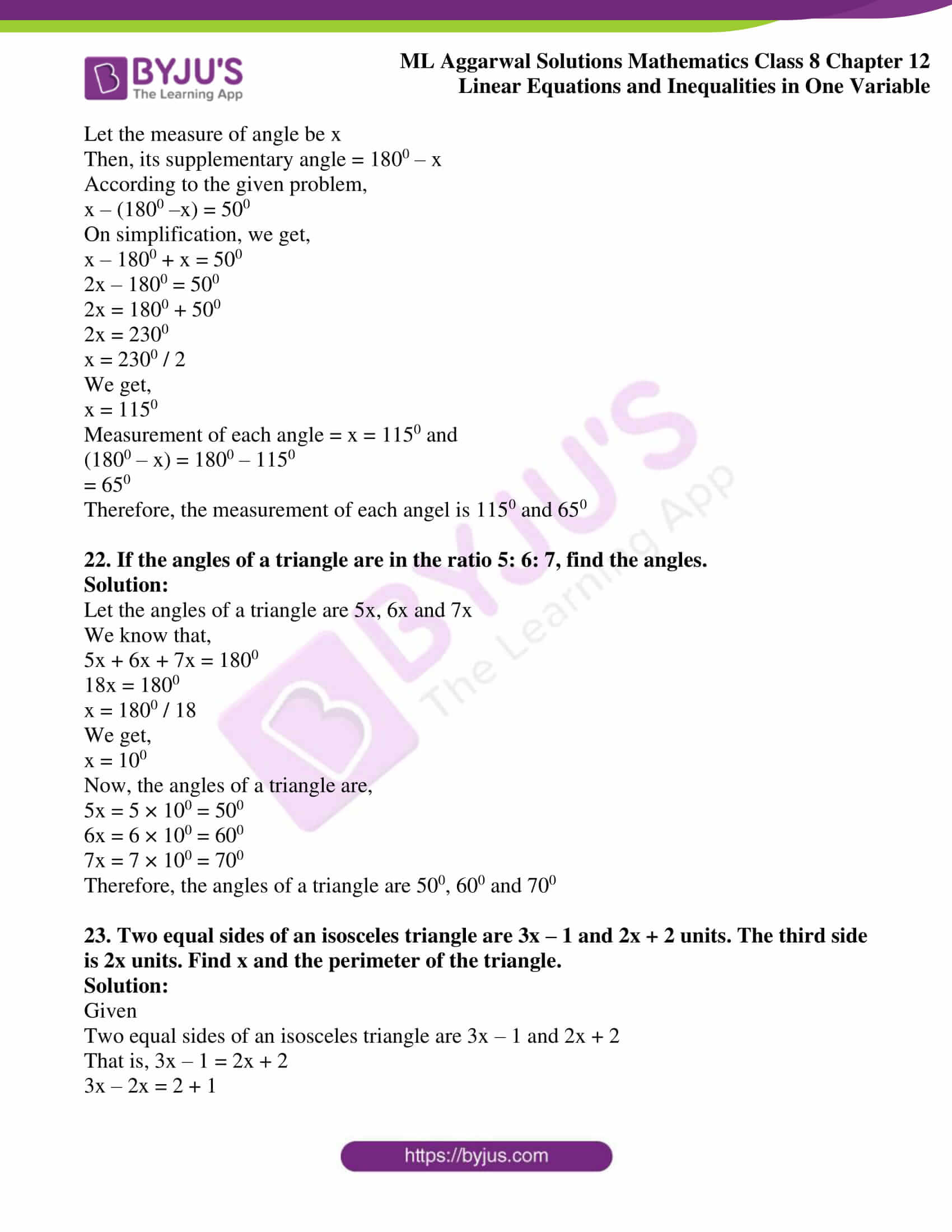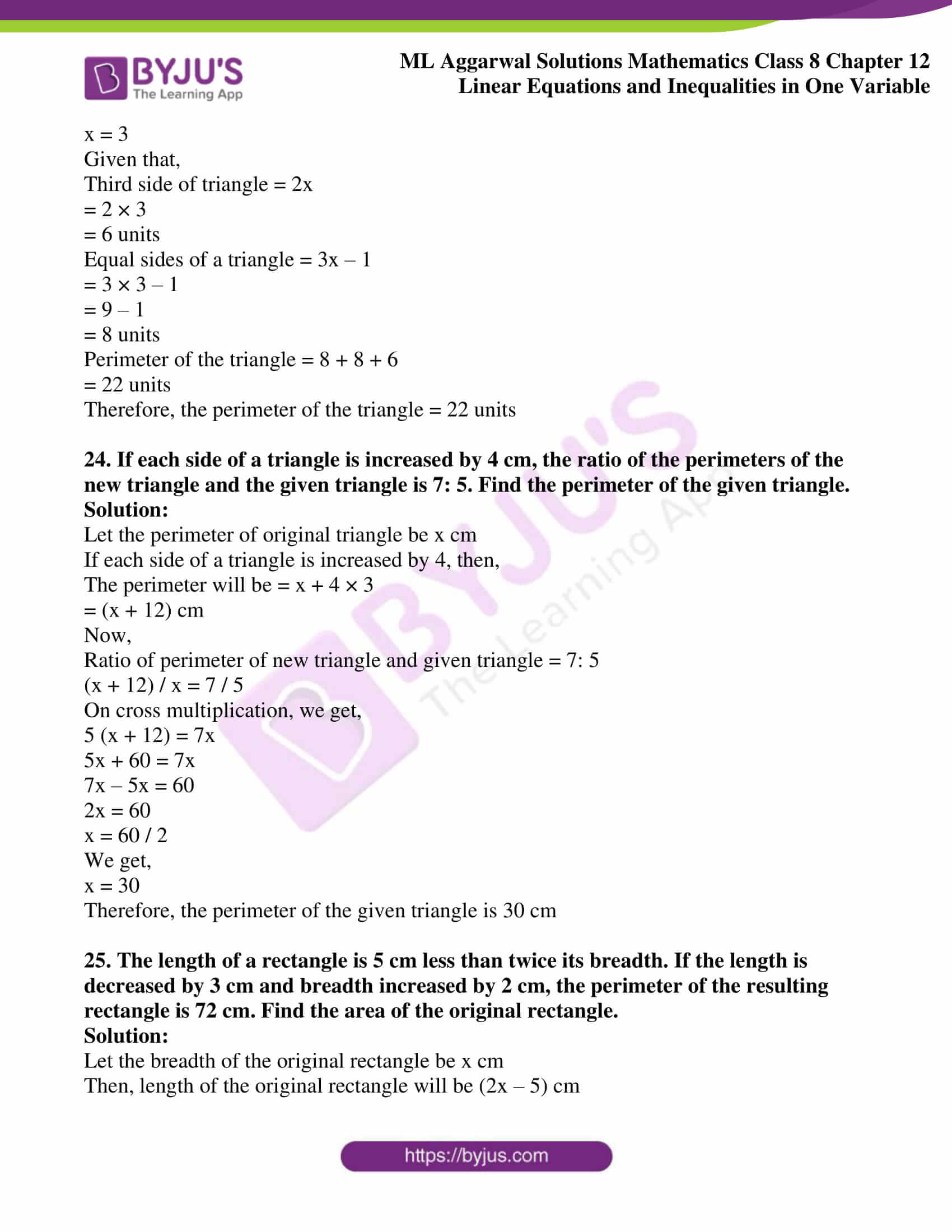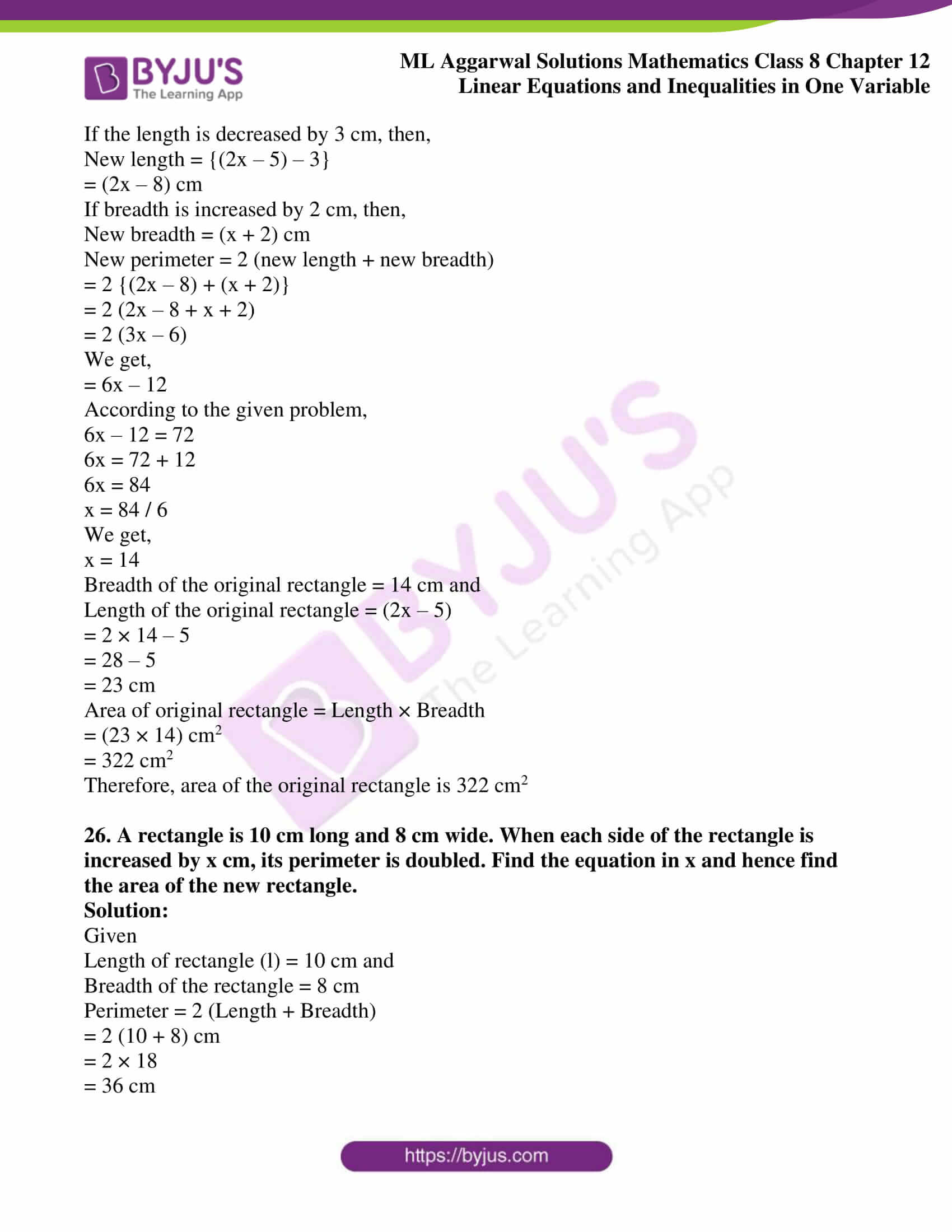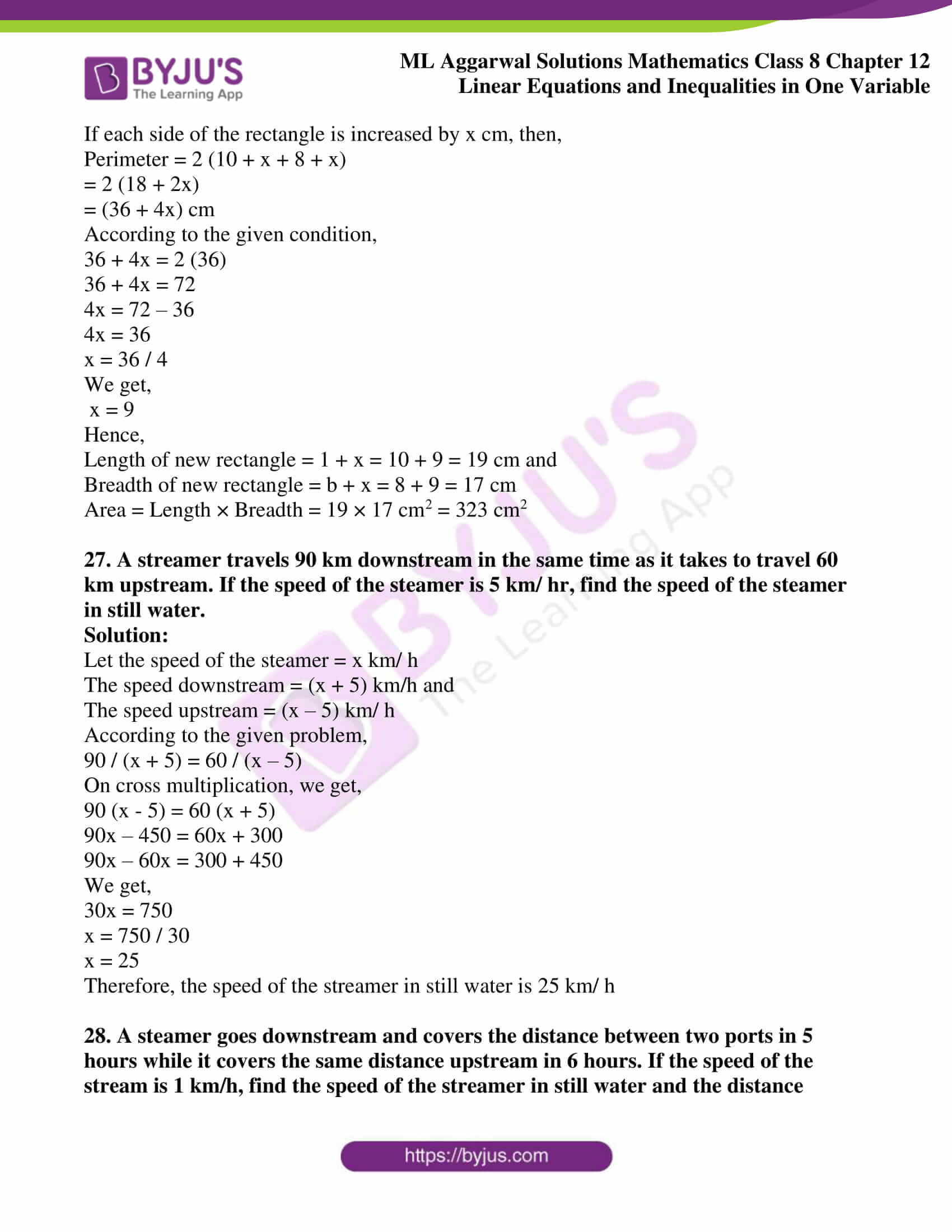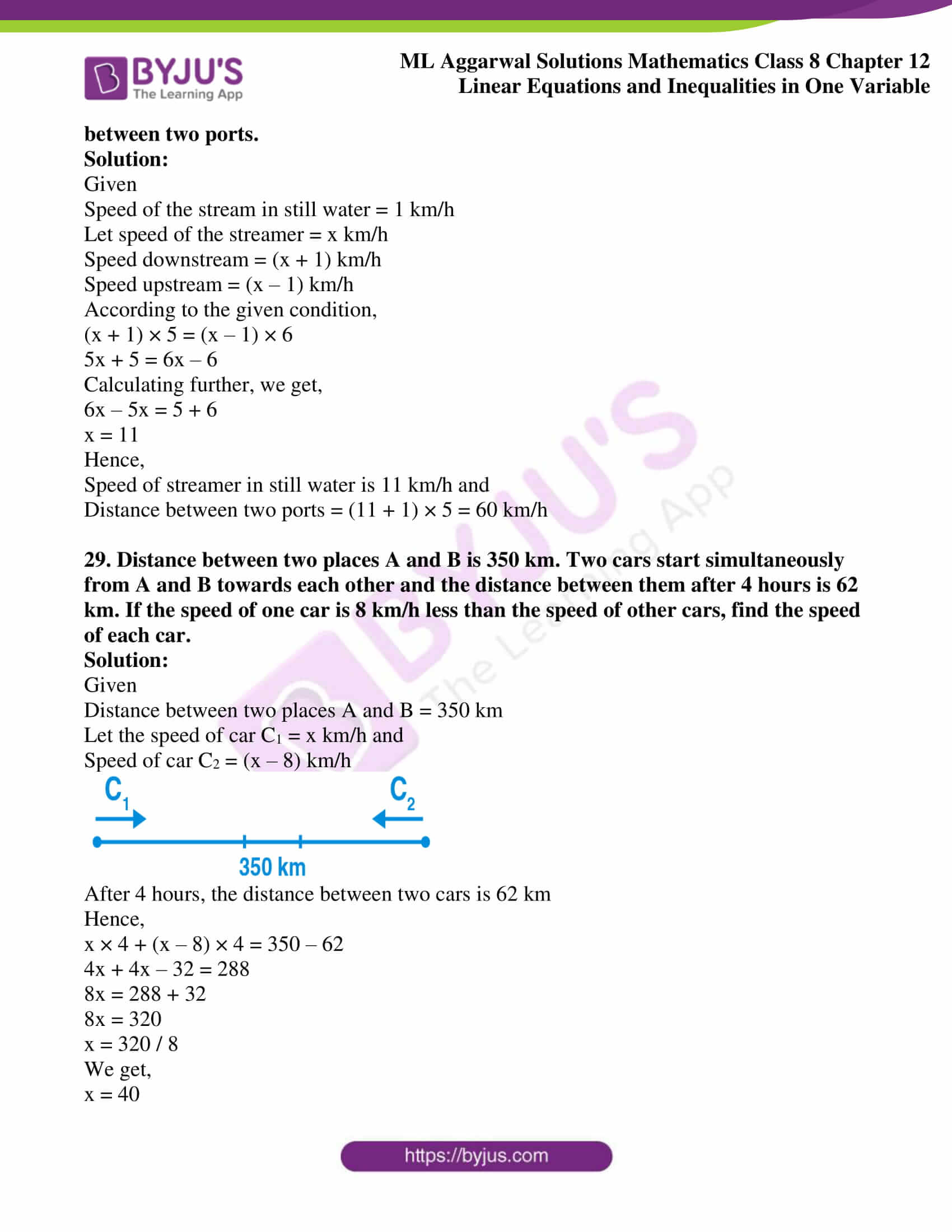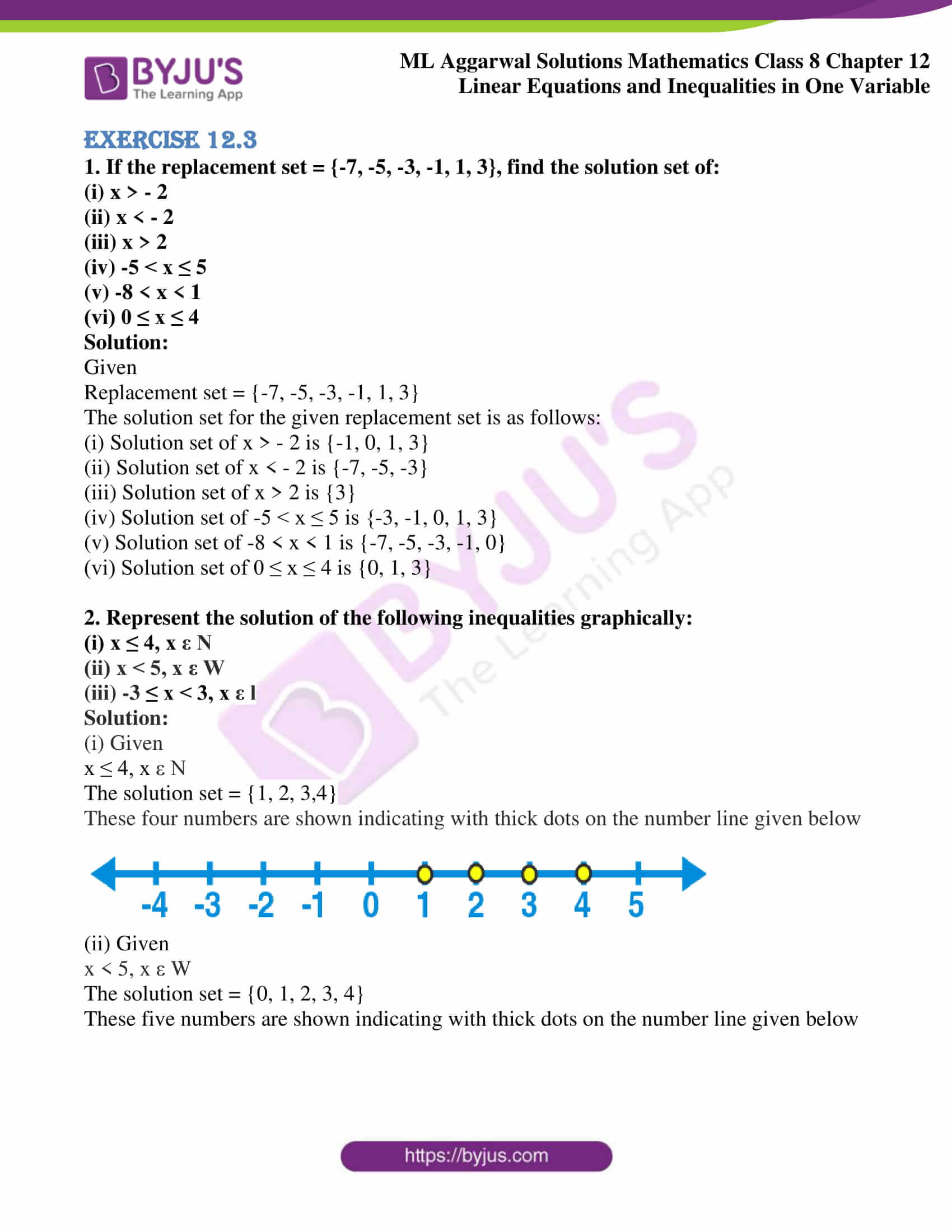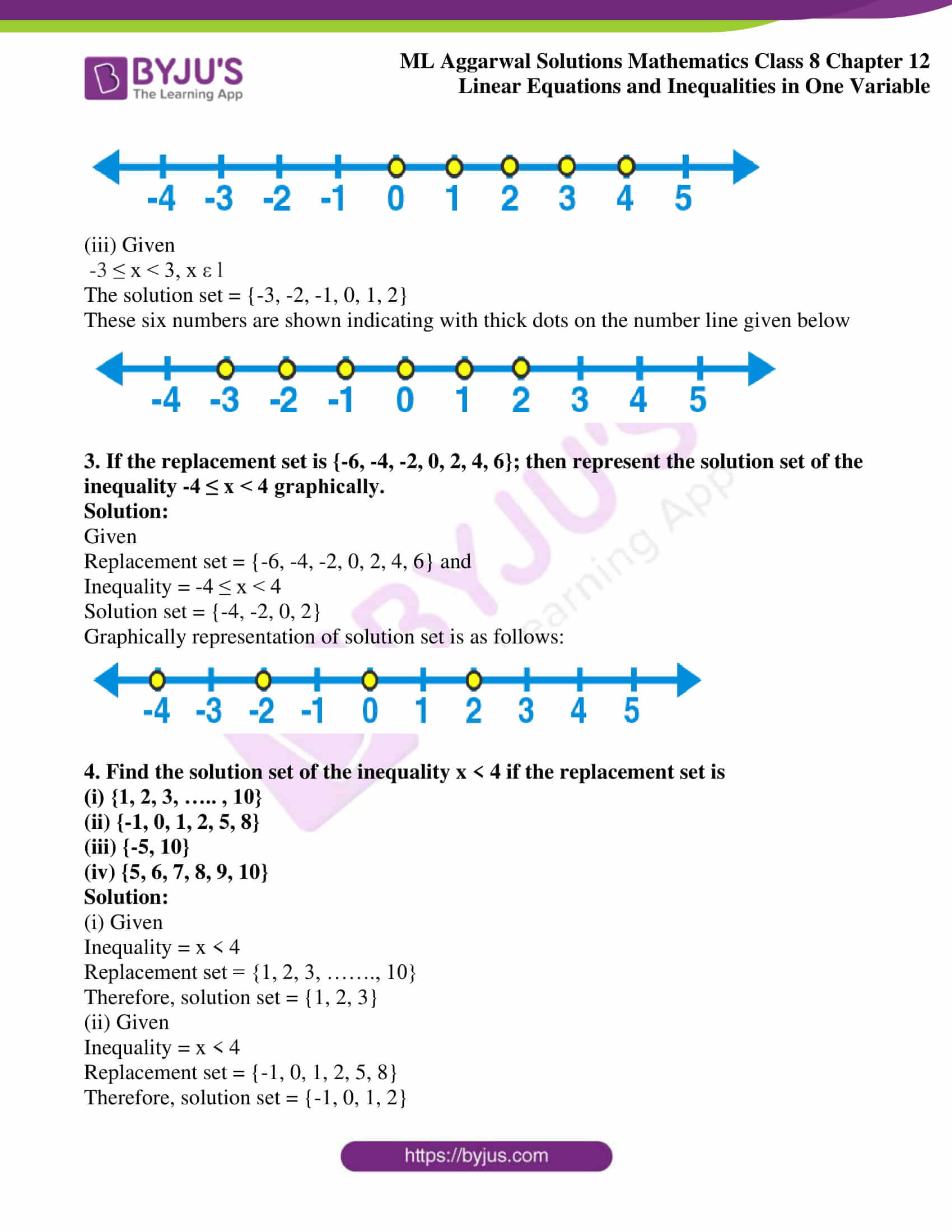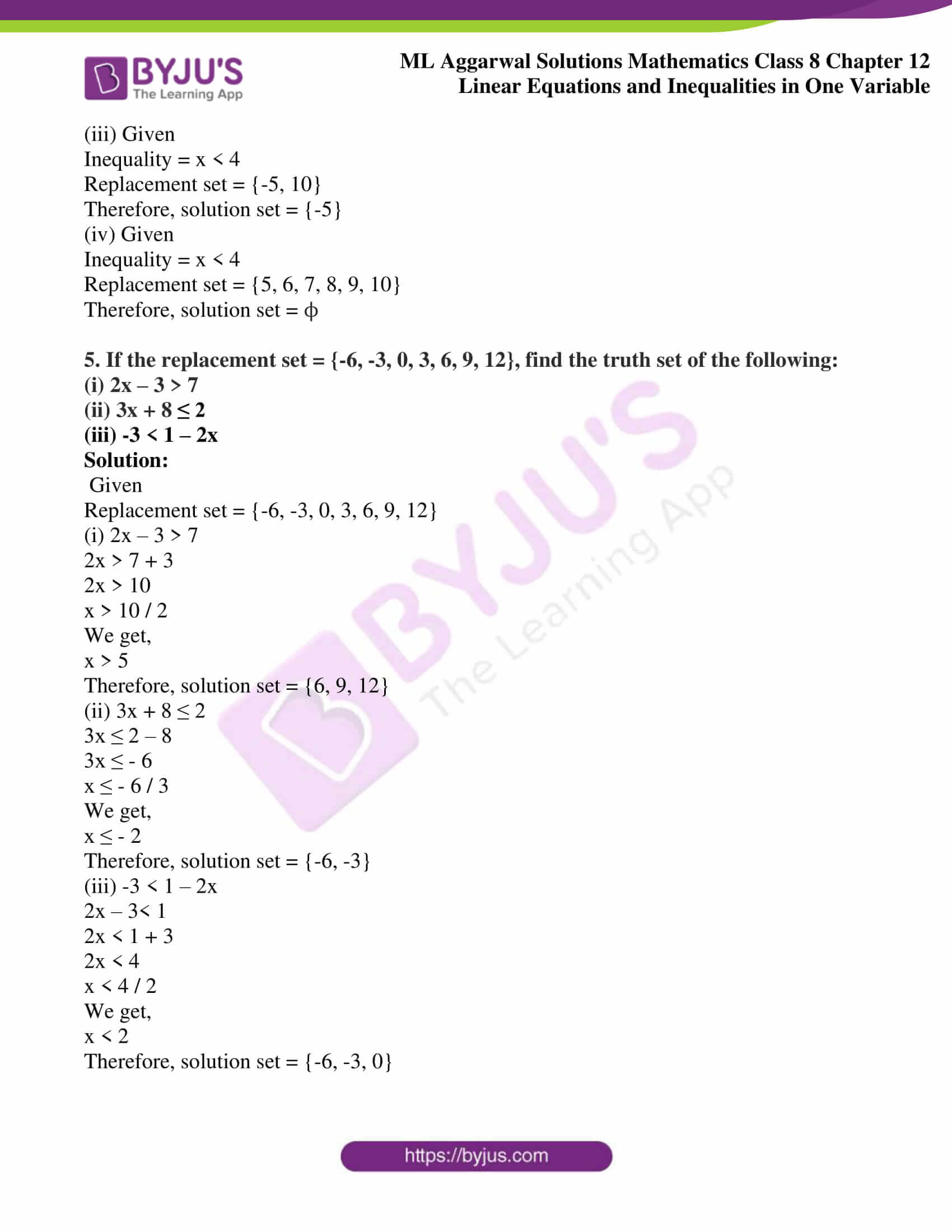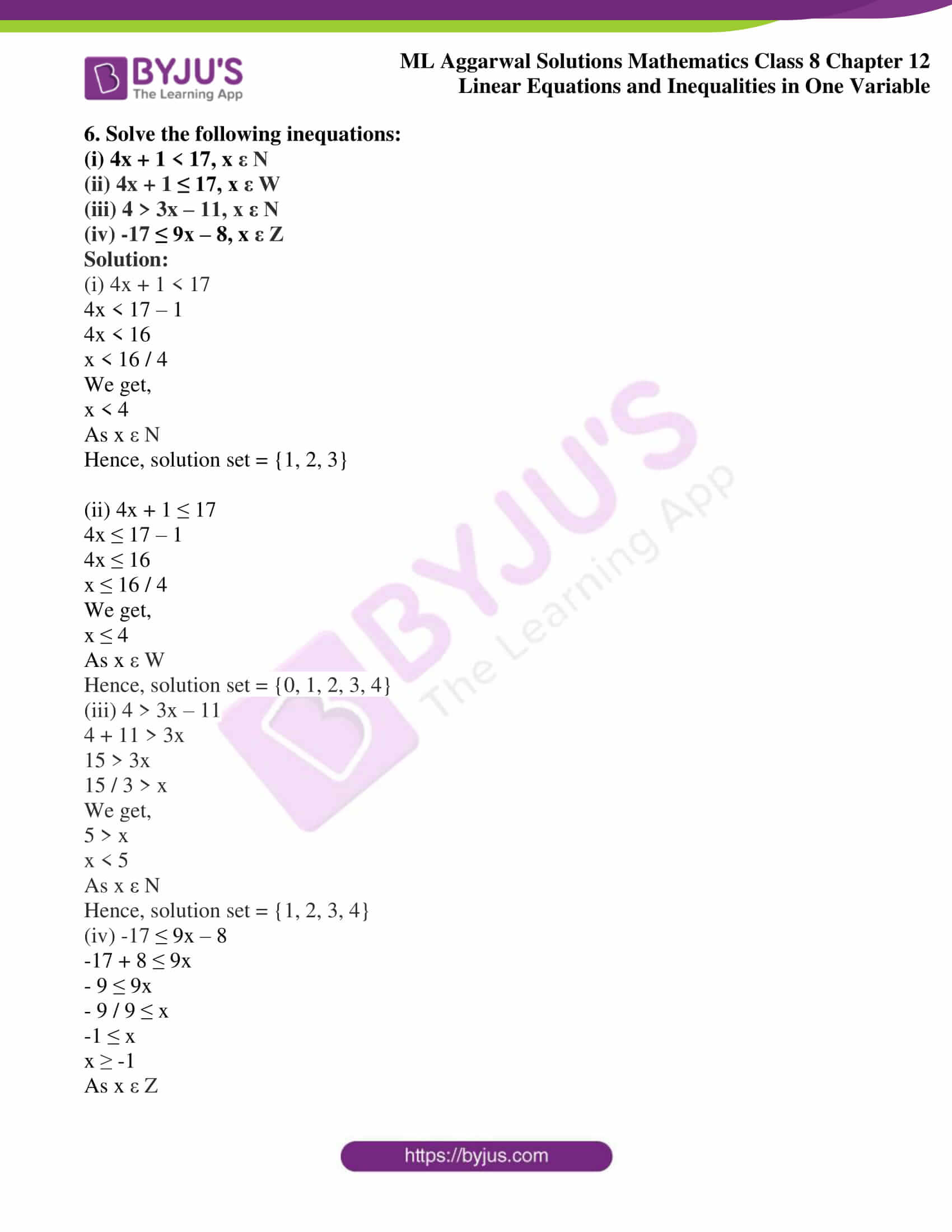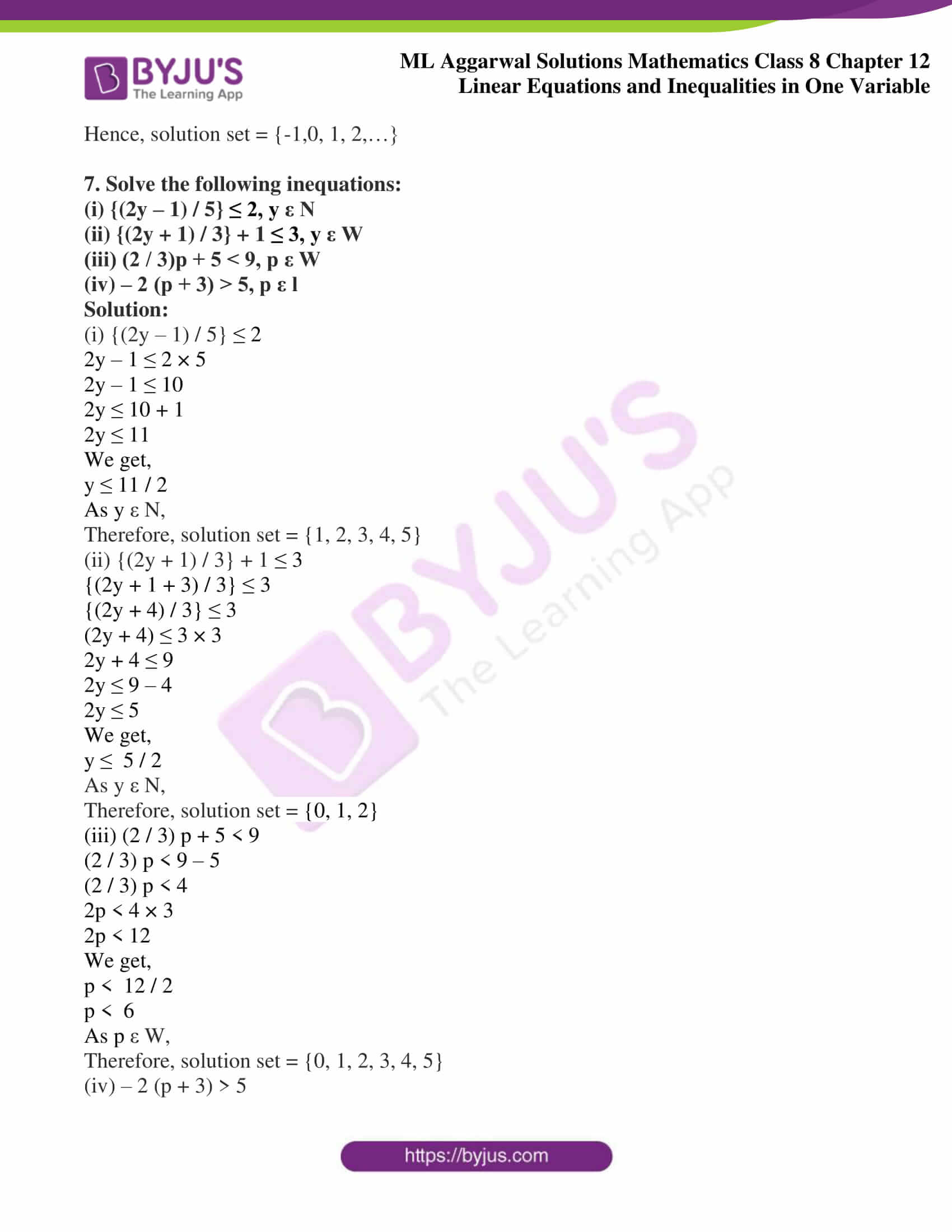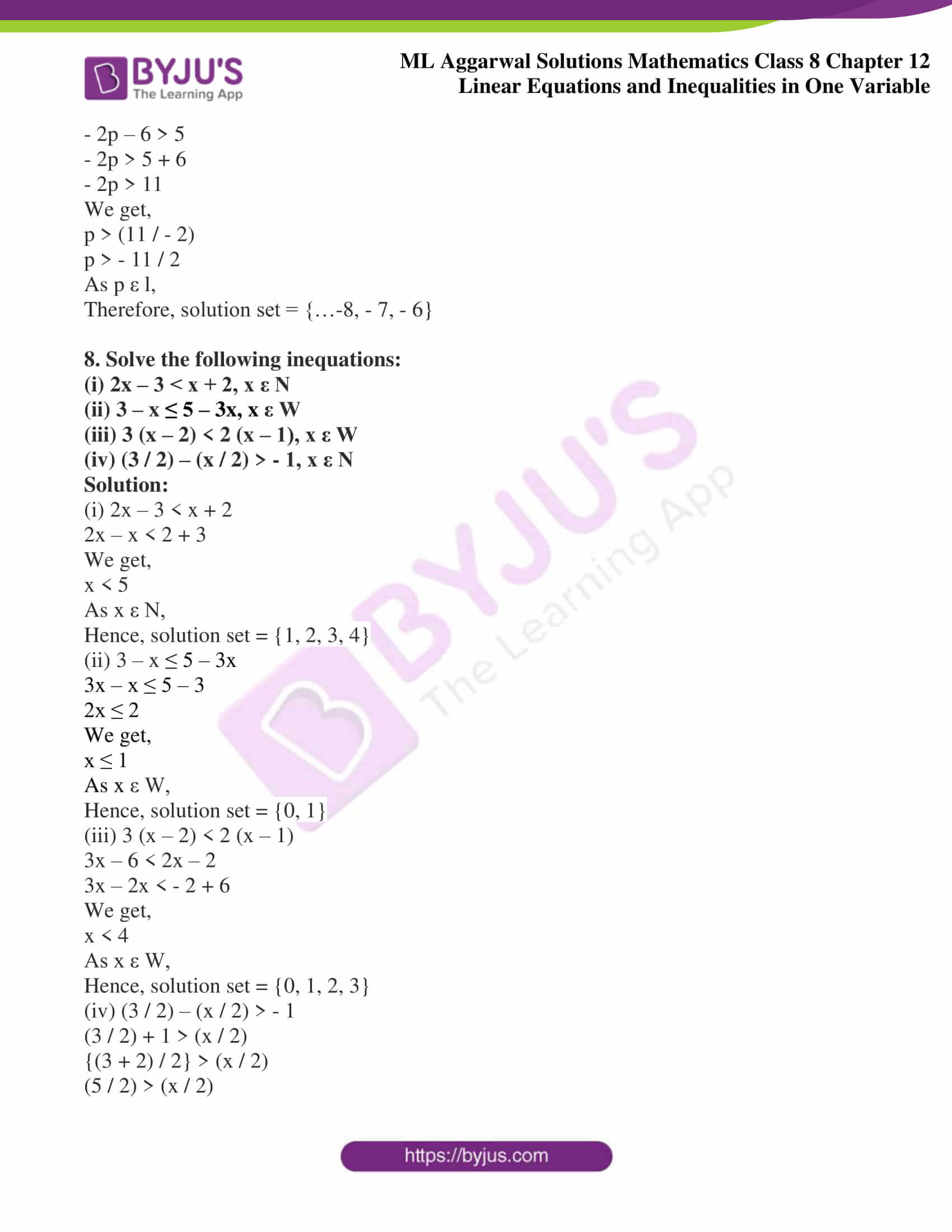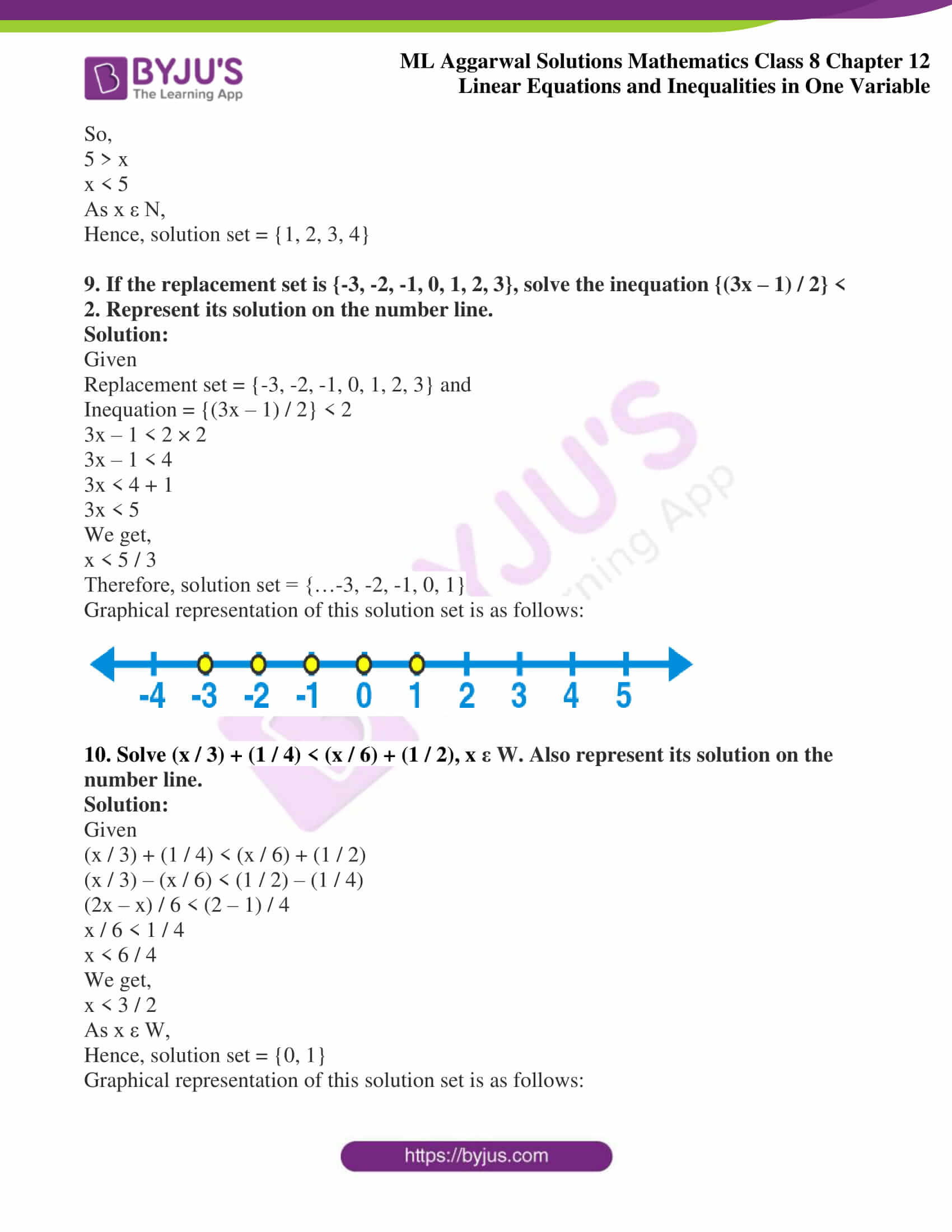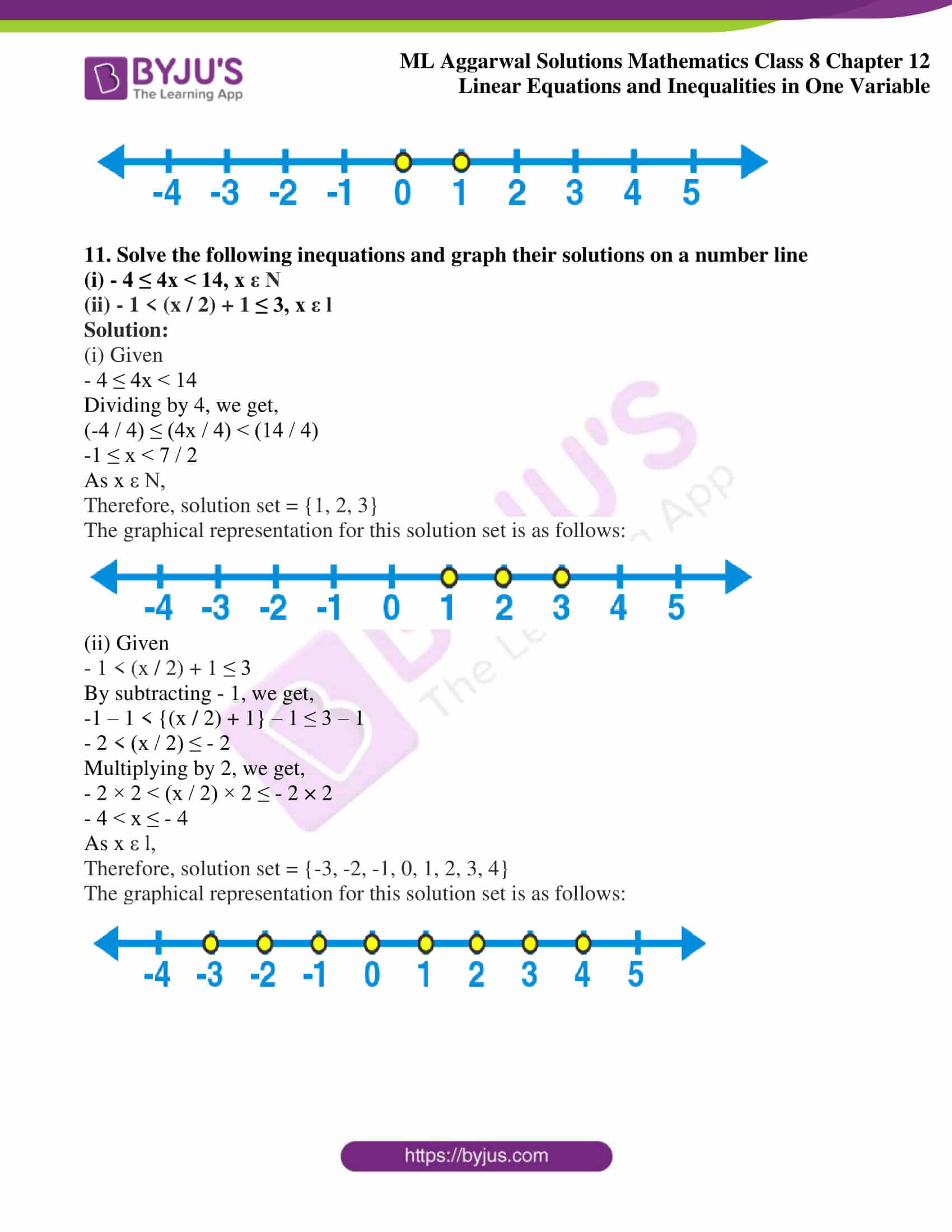## Access ML Aggarwal Solutions for Class 8 Maths Chapter 12 Linear Equations and Inequalities in One Variable

Exercise 12.1

Solve the following equations:

1.

(i) 5x – 3 = 3x – 5

(ii) 3x – 7 = 3(5 – x)

Solution:

(i) 5x – 3 = 3x – 5

5x – 3x = – 5 + 3

2x = – 2

We get,

x = – 2 / 2

x = – 1

(ii) 3x – 7 = 3(5 – x)

3x – 7 = 15 – 3x

3x + 3x = 15 + 7

We get,

6x = 22

x = 22 / 6

x = 11 / 3

2.

(i) 4(2x + 1) = 3(x – 1) + 7

(ii) 3(2p – 1) = 5 – (3p – 2)

Solution:

(i) 4(2x + 1) = 3(x – 1) + 7

8x + 4 = 3x – 3 + 7

8x + 4 = 3x + 4

On further calculation, we get,

8x – 3x = 4 – 4

We get,

x = 0

(ii) 3(2p – 1) = 5 – (3p – 2)

6p – 3 = 5 – 3p + 2

6p + 3p = 5 + 3 + 2

We get,

9p = 10

p = 10 / 9

p =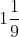3.

(i) 5y – 2{y – 3(y – 5)} = 6

(ii) 0.3(6 – x) = 0.4(x + 8)

Solution:

(i) 5y – 2 {y – 3(y – 5)} = 6

5y – 2 (y – 3y + 15) = 6

5y – 2 (- 2y + 15) = 6

On simplification, we get,

5y + 4y – 30 = 6

5y + 4y = 6 + 30

9y = 36

y = 36 / 9

We get,

y = 4

(ii) 0.3 (6 – x) = 0.4 (x + 8)

1.8 – 0.3x = 0.4x + 3.2

– 0.3x – 0.4x = 3.2 – 1.8

We get,

– 0.7x = 1.4

x = – (1.4 / 0.7)

x = – (14 / 7)

x = – 2

4.

(i) (x – 1) / 3 = {(x + 2) / 6} + 3

(ii) (x + 7) / 3 = 1 + {(3x – 2) / 5}

Solution:

(i) {(x – 1) / 3} = {(x + 2) / 6} + 3

{(x – 1) / 3} – {(x + 2) / 6} = 3

On further calculation, we get,

{2 (x – 1) – 1(x + 2)} / 6 = 3

(2x – 2 – 1x – 2) / 6 = 3

(x – 4) / 6 = 3

We get,

x – 4 = 6 × 3

x – 4 = 18

x = 18 + 4

We get,

x = 22

(ii) (x + 7) / 3 = 1 + {(3x – 2) / 5}

{(x + 7) / 3} – {(3x – 2) / 5} = 1

On further calculation, we get,

{5 (x + 7) – 3 (3x – 2)} / 15 = 1

{(5x + 35) – (9x – 6)} / 15 = 1

(5x – 9x + 35 + 6) / 15 = 1

(- 4x + 41) / 15 = 1

– 4x + 41 = 15

– 4x = 15 – 41

– 4x = – 26

x = – 26 / – 4

We get,

x = 13 / 2

x =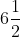5.

(i) {(y + 1) / 3} – {(y – 1) / 2} = (1 + 2y) / 3

(ii) (p / 3) + (p / 4) = 55 – {(p + 40) / 5}

Solution:

(i) {(y + 1) / 3} – {(y – 1) / 2} = (1 + 2y) / 3

{2 (y + 1) – 3 (y – 1)} / 6 = (1 + 2y) / 3

(2y + 2 – 3y + 3) / 6 = (1 + 2y) / 3

We get,

(- y + 5) / 6 = (1 + 2y) / 3

3 (- y + 5) = 6 (1 + 2y)

– 3y + 15 = 6 + 12y

– 3y – 12y = 6 – 15

– 15y = – 9

y = – 9 / – 15

We get,

y = 3 / 5

(ii) (p / 3) + (p / 4) = 55 – {(p + 40) / 5}

(p / 3) + (p / 4) + {(p + 40)} / 5 = 55

Here, L.C.M. of 3, 4, 5 is 60

We get,

{20p +15p + 12(p + 40)} / 60 = 55

(20p + 15p + 12p + 480) / 60 = 55

(47p + 480) / 6 = 55

47p + 480 = 55 × 60

47p + 480 = 3300

47p = 3300 – 480

47p = 2820

p = 2820 / 47

We get,

p = 60

6.

(i) n – {(n – 1) / 2} = 1 – {(n – 2) / 3}

(ii) {(3t – 2) / 3} + {(2t + 3) / 2} = t + (7 / 6)

Solution:

(i) n – {(n – 1) / 2} = 1 – {(n – 2) / 3}

(2n – n + 1) / 2 = (3 – n + 2) / 3

(n + 1) / 2 = (5 – n) / 3

3 (n + 1) = 2 (5 – n)

3n + 3 = 10 – 2n

3n + 2n = 10 – 3

5n = 7

n = 7 / 5

We get,

n =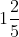(ii) {(3t – 2) / 3} + {(2t + 3) / 2} = t + (7 / 6)

{2 (3t – 2) + 3 (2t + 3)} / 6 = (6t + 7) / 6

(6t – 4) + (6t + 9) = 6t + 7

On further calculation, we get,

6t + 6t + 9 – 4 = 6t + 7

12t + 5 = 6t + 7

12t – 6t = 7 – 5

6t = 2

t = 2 / 6

We get,

t = 1 / 3

7.

(i) 4 (3x + 2) – 5 (6x – 1) = 2 (x – 8) – 6 (7x – 4)

(ii) 3 (5x + 7) + 5 (2x – 11) = 3 (8x – 5) – 15

Solution:

(i) 4 (3x + 2) – 5 (6x – 1) = 2 (x – 8) – 6 (7x – 4)

12x + 8 – 30x + 5 = 2x – 16 – 42x + 24

On further calculation, we get,

– 18x + 13 = – 40x + 8

– 18x + 40x = 8 – 13

We get,

22x = – 5

x = – 5 / 22

(ii) 3 (5x + 7) + 5 (2x – 11) = 3 (8x – 5) – 15

15x + 21 + 10x – 55 = 24x – 15 – 15

On further calculation, we get,

25x – 34 = 24x – 30

25x – 24x = – 30 + 34

We get,

x = 4

8.

(i) (3 – 2x) / (2x + 5) = – (3 / 11)

(ii) (5p + 2) / (8 – 2p) = 7 / 6

Solution:

(i) (3 – 2x) / (2x + 5) = – (3 / 11)

11 (3 – 2x) = – 3 (2x + 5)

On simplification, we get,

33 – 22x = – 6x – 15

– 22x + 6x = – 15 – 33

– 16x = – 48

x = 48 / 16

We get,

x = 3

(ii) (5p + 2) / (8 – 2p) = 7 / 6

6 (5p + 2) = 7 (8 – 2p)

On further calculation, we get,

30p + 12 = 56 – 14p

30p + 14p = 56 – 12

44p = 44

p = 44 / 44

We get,

p = 1

9.

(i) 5 / x = 7 / (x – 4)

(ii) 4 / (2x + 3) = 5 / (x + 4)

Solution:

(i) 5 / x = 7 / (x – 4)

5 (x – 4) = 7x

5x – 20 = 7x

We get,

5x – 7x = 20

– 2x = 20

x = 20 / – 2

x = (- 20 / 2)

We get,

x = – 10

(ii) 4 / (2x + 3) = 5 / (x + 4)

4 (x + 4) = 5 (2x + 3)

4x + 16 = 10x + 15

On further calculation, we get,

4x – 10x = 15 – 16

– 6x = – 1

x = – 1 / – 6

We get,

x = 1 / 6

10.

(i) {(2x + 5) / 2} – {5x / (x – 1)} = x

(ii) 1 / 5 {(1 / 3x) – 5} = 1 / 3 {3 – (1 / x)}

Solution:

(i) {(2x + 5) / 2} – {5x / (x – 1)} = x

{(2x + 5) (x – 1) – (5x) (2)} / {2 (x – 1)} = x

On further calculation, we get,

{2x (x – 1) + 5 (x – 1) – 10x} / (2x – 2) = x

(2x2 – 2x + 5x – 5 – 10x) / (2x – 2) = x

(2x2 – 7x – 5) / (2x – 2) = x

2x2 – 7x – 5 = x (2x – 2)

2x2 – 7x – 5 = 2x2 – 2x

– 7x – 5 = – 2x

– 7x + 2x = 5

– 5x = 5

x = 5 / – 5

We get,

x = – 1

(ii) 1 / 5 {(1 / 3x) – 5} = 1 / 3 {3 – (1 / x)}

1 / 5 [{1 – 5 (3x)} / 3x] = 1 / 3 [{(3x – 1)} / x]

On further calculation, we get,

1 / 5 {(1 – 15x) / 3x} = 1 / 3 {(3x – 1) / x}

We get,

(1 – 15x) / 15x = (3x – 1) / 3x

3x (1 – 15x) = 15x (3x – 1)

3 (1 – 15x) = 15 (3x – 1)

3 – 45x = 45x – 15

– 45x – 45x = – 15 – 3

– 90x = – 18

x = 18 / 90

We get,

x = 1 / 5

11.

(i) {(2x – 3) / (2x – 1)} = {(3x – 1) / (3x + 1)}

(ii) {(2y + 3) / (3y + 2)} = {(4y + 5) / (6y + 7)}

Solution:

(i) {(2x – 3) / (2x – 1)} = {(3x – 1) / (3x + 1)}

(2x – 3) (3x + 1) = (3x – 1) (2x – 1)

On further calculation, we get,

6x2 + 2x – 9x – 3 = 6x2 – 3x – 2x + 1

6x2 – 7x – 3 = 6x2 – 5x + 1

6x2 – 7x – 6x2 + 5x = 1 + 3

– 7x + 5x = 4

– 2x = 4

We get,

x = 4 / – 2

x = – 2

(ii) {(2y + 3) / (3y + 2)} = {(4y + 5) / (6y + 7)}

(2y + 3) (6y + 7) = (4y + 5) (3y + 2)

12y2 + 14y + 18y + 21 = 12y2 + 8y + 15y + 10

On simplification, we get,

32y + 21 = 23y + 10

32y – 23y = 10 – 21

9y = – 11

We get,

y = – 11 / 9

12. If x = p + 1, find the value of p from the equation (1 / 2) (5x – 30) – (1/ 3) (1 + 7p) = 1 / 4

Solution:

Given

x = p + 1 ….. (1)

(1 / 2) (5x – 30) – (1 / 3) (1 + 7p) = 1 / 4 ………. (2)

Substituting the value of x from (1) in (2), we get,

(1 / 2) {5 (p + 1) – 30} – (1 / 3) (1 + 7p) = 1 / 4

1 / 2 (5p + 5 – 30) – 1 / 3 (1 + 7p) = 1 / 4

On further calculation, we get,

1 / 2 (5p – 25) – 1 / 3 (1 + 7p) = 1 / 4

(5p – 25) / 2 – (1 + 7p) / 3 = 1 / 4

{3 (5p – 25) – 2 (1 + 7p)} / 6 = 1 / 4

(15p – 75 – 2 – 14p) / 6 = 1 / 4

(p – 77) / 6 = 1 / 4

On simplification, we get,

4 (p – 77) = 6 (1)

4p – 308 = 6

4p = 6 + 308

4p = 314

p = 314 / 4

We get,

p = 157 / 2

p =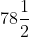13.

Solve {(x + 3) / 3} – {(x – 2) / 2} = 1, Hence find p if (1 / x) + P = 1

Solution:

{(x + 3) / 3} – {(x – 2) / 2} = 1

{2 (x + 3) – 3(x – 2)} / 6 = 1

(2x + 6 – 3x + 6) / 6 = 1

On further calculation, we get,

(- x + 12) / 6 = 1

– x + 12 = 1 × 6

– x + 12 = 6

– x = 6 – 12

– x = – 6

We get,

x = 6

Now,

(1 / x) + P = 1

Substituting x = 6, we get,

(1 / 6) + P = 1

(1 + 6P) / 6 = 1

(1 + 6P) = 6

6P = 6 – 1

6P = 5

P = 5 / 6

Exercise 12.2

1. Three more than twice a number is equal to four less than the number. Find the number.

Solution:

Let the number be x

Twice the number = 2x

As per the given statement,

3 + 2x = x – 4

3 + 2x + 4 = x

We get,

7 = x – 2x

7 = – x

– x = 7

We get,

x = – 7

Therefore, the required number is -7

2. When four consecutive integers are added, the sum is 46. Find the integers.

Solution:

Let the first integer be x, then the next three consecutive integers will be,

(x + 1), (x + 2) and (x + 3)

According to the problem,

x + (x + 1) + (x + 2) + (x + 3) = 46

x + x + 1 + x + 2 + x + 3 = 46

On further calculation, we get,

4x + 6 = 46

4x = 46 – 6

4x = 40

x = 40 / 4

We get,

x = 10

Therefore, four consecutive integers are 10, (10 + 1), (10 + 2) and (10 + 3)

i.e. 10, 11, 12 and 13

3. Manjula thinks a number and subtracts 7 / 3 from it. She multiplies the result by 6. The result now obtained is 2 less than twice the same number she thought of. What is the number?

Solution:

Let a number thought by Manjula be x

According to the statement,

(x – 7 / 3) × 6 = 2x – 2

6x – 14 = 2x – 2

On further calculation, we get,

6x – 2x = – 2 + 14

4x = 12

x = 12 / 4

We get,

x = 3

Therefore, the required number is 3

4. A positive number is 7 times another number. If 15 is added to both the numbers, then one of the new numbers becomes (5 / 2) times the other new number. What are the numbers?

Solution:

Let the required number be x

Then the other number = x / 7

According to the condition,

x + 15 = 5 / 2 {(x / 7) + 15}

2 (x + 15) = (5x / 7) + (5 × 15)

2x + 30 = (5x / 7) + 75

On simplification, we get,

2x – (5 / 7) x = 75 – 30

{(14 – 5) / 7}x = 45

9x / 7 = 45

x = 45 × 7 / 9

We get,

x = 35

Hence,

One number = 35

Other number = 35 / 7 = 5

Therefore, the numbers are 35 and 5

5. When three consecutive even integers are added, the sum is zero. Find the integers.

Solution:

Let the first even integer = x

Then next two consecutive even integers = (x + 2) and (x + 4)

According to the given statement,

x + (x + 2) + (x + 4) = 0

x + x + 2 + x + 4 = 0

3x + 6 = 0

3x = – 6

x = – 6 / 3

We get,

x = – 2

Therefore, three consecutive integers are -2, (- 2 + 2) and (- 2 + 4) i.e. -2, 0 and 2

6. Find two consecutive odd integers such that two-fifth of the smaller exceeds two-ninth of the greater by 4.

Solution:

Let the first odd integer = x

Then next consecutive odd integers = (x + 2)

According to the given problem,

(2 / 5) (x) = (2 / 9) (x + 2) + 4

2x / 5 = {2 (x + 2)} / 9 + 4

On further calculation, we get,

(2x / 5) – {2 (x + 2)} / 9 = 4

{18x – 10 (x + 2)} / 45 = 4

(18x – 10x – 20) / 45 = 4

(8x – 20) / 45 = 4

8x – 20 = 4 × 45

8x – 20 = 180

8x = 180 + 20

8x = 200

x = 200 / 8

We get,

x = 25

So, two consecutive odd integers are x = 25 and

(x + 2) = (25 + 2) = 27

Therefore, two consecutive odd integers are 25 and 27

7. The denominator of a fraction is 1 more than twice its numerator. If the numerator and denominator are both increased by 5, it becomes (3 / 5). Find the original fraction.

Solution:

Let the numerator of the original fraction = x

Then, its denominator = 2x + 1

Hence,

The fraction = x / (2x + 1)

According to the given problem,

(x + 5) / {(2x + 1) + 5} = 3 / 5

(x + 5) / (2x + 1 + 5) = 3 / 5

On further calculation, we get,

5 (x + 5) = 3 (2x + 6)

5x + 25 = 6x + 18

5x – 6x = 18 – 25

-x = – 7

We get,

x = 7

Now,

Original fraction = x / (2x + 1)

= 7 / {2 (7) + 1}

= 7 / (14 + 1)

We get,

= 7 / 15

Therefore, the original fraction is 7 / 15

8. Find two positive numbers in the ratio 2: 5 such that their difference is 15.

Solution:

Let the two numbers be 2x and 5x

Because the ratio of these two numbers = 2x / 5x

= 2 / 5

= 2: 5

According to the given problem,

5x – 2x = 15

3x = 15

x = 15 / 3

We get,

x = 5

So, the numbers are 2x = 2 × 5 = 10 and 5x = 5 × 5 = 25

Therefore the required numbers are 10 and 25

9. What number should be added to each of the numbers 12, 22, 42 and 72 so that the resulting numbers may be in proportion?

Solution:

Let x be the required number

According to the given problem,

(12 + x), (22 + x), (42 + x) and (72 + x) are in proportion

So,

(12 + x) / (22 + x) = (42 + x) / (72 + x)

On cross multiplication, we get,

(12 + x) (72 + x) = (42 + x) (22 + x)

On simplification, we get,

12 (72 + x) + x (72 + x) = 42 (22 + x) + x (22 + x)

864 + 12x + 72x + x2 = 924 + 42x + 22x + x2

We get,

864 + 84x + x2 = 924 + 64x + x2

864 + 84x + x2 – 924 – 64x – x2 = 0

864 + 84x – 64x – 924 = 0

84x – 64x = 924 – 864

20x = 60

We get,

x = 60 / 20

x = 3

Therefore, the required number is 3

10. The digits of a two-digit number differ by 3. If the digits are interchanged and the resulting number is added to the original number, we get 143. What can be the original number?

Solution:

Let one’s digit of a two-digit number be x

Given that the difference between both the digits is 3,

Then ten’s digit = x + 3

Hence, the number = x + 10 (x + 3)

= x + 10x + 30

= 11x + 30

By interchanging the digits, we get,

One’s digit of a new number = x + 3 and

Ten’s digit of a new number = x

Hence,

Number = x + 3 + 10x = 11x + 3

According to the condition,

11x + 30 + 11x + 3 = 143

22x + 33 = 143

22x = 143 – 33

We get,

22x = 110

x = 110 / 22

x = 5

Therefore, original number = 11x + 30

= 11 × 5 + 30

= 55 + 30

We get,

= 85

Hence, the original number is 85

11. Sum of the digits of a two-digit number is 11. When we interchange the digits, it is found that the resulting new number is greater than the original number by 63. Find the two- digit number.

Solution:

Given

Sum of the digits of a two-digit numbers = 11

Let unit’s digit of a 2-digit number be x

Then ten’s digit will be 11 – x

So, number = x + 10 (11 – x)

= x + 110 – 10x

= 110 – 9x

Now, by interchanging the digits, we get,

One’s digit of a new number = 11 – x

And ten’s digit will be = x

Hence, number = 11 – x + 10x

= 11 + 9x

According to the condition,

11 + 9x – (110 – 9x) = 63

11 + 9x – 110 + 9x = 63

18x = 63 – 11 + 110

18x = 162

We get,

x = 162 / 18

x = 9

So, original number = 110 – 9x

= 110 – 9 × 9

= 110 – 81

= 29

Therefore, the original number is 29

12. Ritu is now four times as old as his brother Raju. In 4 years time, her age will be twice of Raju’s age. What are their present ages?

Solution:

Let the age of Raju be x years

Then the age of Ritu will be = 4 × x years

In 4 years time,

Age of Raju = (x + 4) years

Age of Ritu = (4x + 4) years

According to the given problem,

4x + 4 = 2 (x + 4)

4x + 4 = 2x + 8

4x – 2x = 8 – 4

2x = 4

We get,

x = 4 / 2

x = 2

Therefore, Raju’s age = 2 years and

Ritu’s age = 4 × 2 = 8 years

13. A father is 7 times as old as his son. Two years ago, the father was 13 times as old as his son. How old are they now?

Solution:

Let the present age of son be x years

Then, age of his father will be 7x years

Two years ago, age of son = (x – 2) years

Two years ago, age of his father = (7x – 2) years

According to the given problem,

7x – 2 = 13 (x – 2)

7x – 2 = 13x – 26

7x – 13x = – 26 + 2

– 6x = – 24

x = – 24 /- 6

We get,

x = 4

Therefore, age of son = 4 years and

Age of his father = 7x = 7 × 4 = 28 years

14. The ages of Sona and Sonali are in the ratio 5: 3. Five years hence, the ration of their ages will be 10: 7. Find their present ages.

Solution:

Given

Ratio of ages of Sona and Sonali = 5: 3

Let us consider the present age of Sona and Sonali be 5x and 3x years respectively

Five years hence,

The age of Sona = 5x + 5 and

The age of Sonali = 3x + 5

According to the given problem,

(5x + 5) / (3x + 5) = 10 / 7

On cross multiplication, we get,

7 (5x + 5) 10 (3x + 5)

35x + 35 = 30x + 50

35x – 30x = 50 – 35

5x = 15

x = 15 / 5

We get,

x = 3

Now,

Present age of Sona = 5x = 5 × 3 = 15 years and

Present age of Sonali = 3x = 3 × 3 = 9 years

Therefore, the present age of Sona and Sonali is 15years and 9 years

15. An employee works in a company on a contract of 30 days on the condition that he will receive Rs 200 for each day he works and he will be fined Rs 20 for each day if he is absent. If he receives Rs 3800 in all, for how many days did he remain absent?

Solution:

Given

Period of contract = 30 days

If an employee works a day, he will get = Rs 200

If he is absent, he will be fined = Rs 20 per day

At the end of contract period, he gets = Rs 3800

Let an employee remain absent for x days

Then, number of days, he worked = (30 – x) days

According to the given condition,

(30 – x) × 200 – x × 20 = 3800

6000 – 200x – 20x = 3800

6000 – 220x = 3800

220x = 6000 – 3800

220x = 2200

x = 2200 / 220

We get,

x = 10

Therefore, an employee remained absent for 10 days.

16. I have a total of Rs 300 in coins of denomination Rs 1, Rs 2 and Rs 5. The number of coins is 3 times the number of Rs 5 coins. The total number of coins is 160. How many coins of each denomination are with me?

Solution:

Given

Amount of coins = Rs 300

Total number of coins = 160

Le t the number of coins of Rs 5 = x

Then number of coins of Rs 2 = 3x

And number of coins of Rs 1 = 160 – (x + 3x)

= 160 – 4x

According to the given problem,

(160 – 4x) × 1 + 3x × 2 + x × 5 = 300

160 – 4x + 6x + 5x = 300

We get,

160 + 7x = 300

7x = 300 – 160

7x = 140

x = 140 / 7

We get,

x = 20

Hence, 5 Rs coins = 20

2 Rs coins = 3x = 3 × 20 = 60 and

1 Rs coins = 160 – 60 – 20 = 80

17. A local bus is carrying 40 passengers, some with Rs 5 tickets and the remaining with Rs 7.50 tickets. If the total receipts from these passengers are Rs 230, find the number of passengers with Rs 5 tickets.

Solution:

Let the number of passengers with Rs 5 tickets = x

Then, the number of passengers with Rs 7.50 tickets = (40 – x)

According to the given statement,

5 × x + (40 – x) × 7.50 = 230

On simplification, we get,

5x + 300 – 7.5x = 230

5x – 7.5x = 230 – 300

-2.5x = – 70

x = -70 / – 2.5

We get,

x = 28

Therefore, the number of passengers with Rs 5 tickets = 28

18. On a school picnic, a group of students agree to pay equally for the use of a full boat and pay Rs 10 each. If there had been 3 more students in the group, each would have paid Rs 2 less. How many students were there in the group?

Solution:

Let the number of students in a group be x

If there are 3 more students in a group, then,

The total number of students in a group = x + 3

According to the given problem,

10 × x = (x + 3) × (10 – 2)

On simplification, we get,

10x = (x + 3) × 8

10x = 8 (x + 3)

10x = 8x + 24

10x – 8x = 24

2x = 24

x = 24 / 2

We get,

x = 12

Therefore, the total number of students in the group = 12

19. Half of a herd of deer are grazing in the field and three-fourths of the remaining are playing nearby. The rest 9 are drinking water from the pond. Find the number of deer in the herd.

Solution:

Let the number of deer in the herd = x

Number of deer grazing in the field = x / 2

Remaining = x – (x / 2)

= x / 2

Given that the (3 / 4) of the remaining deer are playing

= (3 / 4) × (1 / 2) x

We get,

= (3 / 8) x

Hence,

Rest of deer = (x / 2) – (3 / 8) x

= (1 / 8) x

Therefore,

(1 / 8) x = 9

x = 9 × 8

We get,

x = 72

Hence, total number of deer in the herd = 72

20. Sakshi takes some flowers in a basket and visits three temples one by one. At each temple, she offers one-half of the flowers from the basket. If she is left with 6 flowers at the end, find the number of flowers she had in the beginning.

Solution:

Let the total number of flowers in the basket = x

Flowers offered in first temple = x / 2

Remaining flowers = x – (x / 2)

= (x / 2)

Flowers offered in the second temple

(x / 2) × (1 / 2) = x / 4

Remaining flowers = (x / 2) – (x / 4)

= (x / 4)

Flowers offered in the third temple = (x / 4) × (1 / 2)

= (x / 8)

Remaining flowers = (x / 4) – (x / 8)

= (x / 8)

Hence,

(x / 8) = 6

We get,

x = 6 × 8

x = 48

Therefore, number of flowers she had in the beginning = 48

21. Two supplementary angles differ by 500. Find the measure of each angle.

Solution:

Let the measure of angle be x

Then, its supplementary angle = 1800 – x

According to the given problem,

x – (1800 –x) = 500

On simplification, we get,

x – 1800 + x = 500

2x – 1800 = 500

2x = 1800 + 500

2x = 2300

x = 2300 / 2

We get,

x = 1150

Measurement of each angle = x = 1150 and

(1800 – x) = 1800 – 1150

= 650

Therefore, the measurement of each angel is 1150 and 650

22. If the angles of a triangle are in the ratio 5: 6: 7, find the angles.

Solution:

Let the angles of a triangle are 5x, 6x and 7x

We know that,

5x + 6x + 7x = 1800

18x = 1800

x = 1800 / 18

We get,

x = 100

Now, the angles of a triangle are,

5x = 5 × 100 = 500

6x = 6 × 100 = 600

7x = 7 × 100 = 700

Therefore, the angles of a triangle are 500, 600 and 700

23. Two equal sides of an isosceles triangle are 3x – 1 and 2x + 2 units. The third side is 2x units. Find x and the perimeter of the triangle.

Solution:

Given

Two equal sides of an isosceles triangle are 3x – 1 and 2x + 2

That is, 3x – 1 = 2x + 2

3x – 2x = 2 + 1

x = 3

Given that,

Third side of triangle = 2x

= 2 × 3

= 6 units

Equal sides of a triangle = 3x – 1

= 3 × 3 – 1

= 9 – 1

= 8 units

Perimeter of the triangle = 8 + 8 + 6

= 22 units

Therefore, the perimeter of the triangle = 22 units

24. If each side of a triangle is increased by 4 cm, the ratio of the perimeters of the new triangle and the given triangle is 7: 5. Find the perimeter of the given triangle.

Solution:

Let the perimeter of original triangle be x cm

If each side of a triangle is increased by 4, then,

The perimeter will be = x + 4 × 3

= (x + 12) cm

Now,

Ratio of perimeter of new triangle and given triangle = 7: 5

(x + 12) / x = 7 / 5

On cross multiplication, we get,

5 (x + 12) = 7x

5x + 60 = 7x

7x – 5x = 60

2x = 60

x = 60 / 2

We get,

x = 30

Therefore, the perimeter of the given triangle is 30 cm

25. The length of a rectangle is 5 cm less than twice its breadth. If the length is decreased by 3 cm and breadth increased by 2 cm, the perimeter of the resulting rectangle is 72 cm. Find the area of the original rectangle.

Solution:

Let the breadth of the original rectangle be x cm

Then, length of the original rectangle will be (2x – 5) cm

If the length is decreased by 3 cm, then,

New length = {(2x – 5) – 3}

= (2x – 8) cm

If breadth is increased by 2 cm, then,

New breadth = (x + 2) cm

New perimeter = 2 (new length + new breadth)

= 2 {(2x – 8) + (x + 2)}

= 2 (2x – 8 + x + 2)

= 2 (3x – 6)

We get,

= 6x – 12

According to the given problem,

6x – 12 = 72

6x = 72 + 12

6x = 84

x = 84 / 6

We get,

x = 14

Breadth of the original rectangle = 14 cm and

Length of the original rectangle = (2x – 5)

= 2 × 14 – 5

= 28 – 5

= 23 cm

Area of original rectangle = Length × Breadth

= (23 × 14) cm2

= 322 cm2

Therefore, area of the original rectangle is 322 cm2

26. A rectangle is 10 cm long and 8 cm wide. When each side of the rectangle is increased by x cm, its perimeter is doubled. Find the equation in x and hence find the area of the new rectangle.

Solution:

Given

Length of rectangle (l) = 10 cm and

Breadth of the rectangle = 8 cm

Perimeter = 2 (Length + Breadth)

= 2 (10 + 8) cm

= 2 × 18

= 36 cm

If each side of the rectangle is increased by x cm, then,

Perimeter = 2 (10 + x + 8 + x)

= 2 (18 + 2x)

= (36 + 4x) cm

According to the given condition,

36 + 4x = 2 (36)

36 + 4x = 72

4x = 72 – 36

4x = 36

x = 36 / 4

We get,

x = 9

Hence,

Length of new rectangle = 1 + x = 10 + 9 = 19 cm and

Breadth of new rectangle = b + x = 8 + 9 = 17 cm

Area = Length × Breadth = 19 × 17 cm2 = 323 cm2

27. A streamer travels 90 km downstream in the same time as it takes to travel 60 km upstream. If the speed of the steamer is 5 km/ hr, find the speed of the steamer in still water.

Solution:

Let the speed of the steamer = x km/ h

The speed downstream = (x + 5) km/h and

The speed upstream = (x – 5) km/ h

According to the given problem,

90 / (x + 5) = 60 / (x – 5)

On cross multiplication, we get,

90 (x – 5) = 60 (x + 5)

90x – 450 = 60x + 300

90x – 60x = 300 + 450

We get,

30x = 750

x = 750 / 30

x = 25

Therefore, the speed of the streamer in still water is 25 km/ h

28. A steamer goes downstream and covers the distance between two ports in 5 hours while it covers the same distance upstream in 6 hours. If the speed of the stream is 1 km/h, find the speed of the streamer in still water and the distance between two ports.

Solution:

Given

Speed of the stream in still water = 1 km/h

Let speed of the streamer = x km/h

Speed downstream = (x + 1) km/h

Speed upstream = (x – 1) km/h

According to the given condition,

(x + 1) × 5 = (x – 1) × 6

5x + 5 = 6x – 6

Calculating further, we get,

6x – 5x = 5 + 6

x = 11

Hence,

Speed of streamer in still water is 11 km/h and

Distance between two ports = (11 + 1) × 5 = 60 km/h

29. Distance between two places A and B is 350 km. Two cars start simultaneously from A and B towards each other and the distance between them after 4 hours is 62 km. If the speed of one car is 8 km/h less than the speed of other cars, find the speed of each car.

Solution:

Given

Distance between two places A and B = 350 km

Let the speed of car C1 = x km/h and

Speed of car C2 = (x – 8) km/h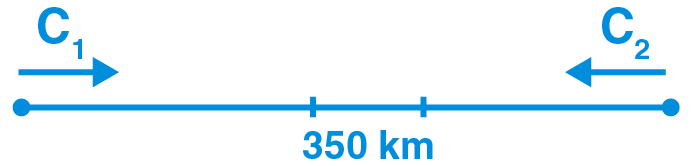After 4 hours, the distance between two cars is 62 km

Hence,

x × 4 + (x – 8) × 4 = 350 – 62

4x + 4x – 32 = 288

8x = 288 + 32

8x = 320

x = 320 / 8

We get,

x = 40

Therefore, speed of car C1 = 40 km/h

Speed of car C2 = (x – 8) = (40 – 8) = 32 km/h

Exercise 12.3

1. If the replacement set = {-7, -5, -3, -1, 1, 3}, find the solution set of:

(i) x > – 2

(ii) x < – 2

(iii) x > 2

(iv) -5 < x ≤ 5

(v) -8 < x < 1

(vi) 0 ≤ x ≤ 4

Solution:

Given

Replacement set = {-7, -5, -3, -1, 1, 3}

The solution set for the given replacement set is as follows:

(i) Solution set of x > – 2 is {-1, 0, 1, 3}

(ii) Solution set of x < – 2 is {-7, -5, -3}

(iii) Solution set of x > 2 is {3}

(iv) Solution set of -5 < x ≤ 5 is {-3, -1, 0, 1, 3}

(v) Solution set of -8 < x < 1 is {-7, -5, -3, -1, 0}

(vi) Solution set of 0 ≤ x ≤ 4 is {0, 1, 3}

2. Represent the solution of the following inequalities graphically:

(i) x ≤ 4, x ε N

(ii) x < 5, x ε W

(iii) -3 ≤ x < 3, x ε l

Solution:

(i) Given

x ≤ 4, x ε N

The solution set = {1, 2, 3,4}

These four numbers are shown indicating with thick dots on the number line given below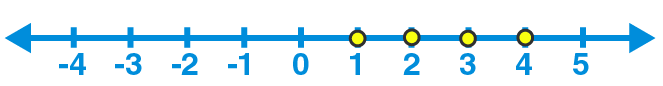(ii) Given

x < 5, x ε W

The solution set = {0, 1, 2, 3, 4}

These five numbers are shown indicating with thick dots on the number line given below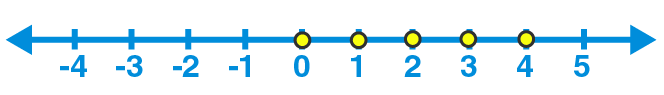(iii) Given

-3 ≤ x < 3, x ε l

The solution set = {-3, -2, -1, 0, 1, 2}

These six numbers are shown indicating with thick dots on the number line given below3. If the replacement set is {-6, -4, -2, 0, 2, 4, 6}; then represent the solution set of the inequality -4 ≤ x < 4 graphically.

Solution:

Given

Replacement set = {-6, -4, -2, 0, 2, 4, 6} and

Inequality = -4 ≤ x < 4

Solution set = {-4, -2, 0, 2}

Graphically representation of solution set is as follows:4. Find the solution set of the inequality x < 4 if the replacement set is

(i) {1, 2, 3, ….. , 10}

(ii) {-1, 0, 1, 2, 5, 8}

(iii) {-5, 10}

(iv) {5, 6, 7, 8, 9, 10}

Solution:

(i) Given

Inequality = x < 4

Replacement set = {1, 2, 3, ……., 10}

Therefore, solution set = {1, 2, 3}

(ii) Given

Inequality = x < 4

Replacement set = {-1, 0, 1, 2, 5, 8}

Therefore, solution set = {-1, 0, 1, 2}

(iii) Given

Inequality = x < 4

Replacement set = {-5, 10}

Therefore, solution set = {-5}

(iv) Given

Inequality = x < 4

Replacement set = {5, 6, 7, 8, 9, 10}

Therefore, solution set = ϕ

5. If the replacement set = {-6, -3, 0, 3, 6, 9, 12}, find the truth set of the following:

(i) 2x – 3 > 7

(ii) 3x + 8 ≤ 2

(iii) -3 < 1 – 2x

Solution:

Given

Replacement set = {-6, -3, 0, 3, 6, 9, 12}

(i) 2x – 3 > 7

2x > 7 + 3

2x > 10

x > 10 / 2

We get,

x > 5

Therefore, solution set = {6, 9, 12}

(ii) 3x + 8 ≤ 2

3x ≤ 2 – 8

3x ≤ – 6

x ≤ – 6 / 3

We get,

x ≤ – 2

Therefore, solution set = {-6, -3}

(iii) -3 < 1 – 2x

2x – 3< 1

2x < 1 + 3

2x < 4

x < 4 / 2

We get,

x < 2

Therefore, solution set = {-6, -3, 0}

6. Solve the following inequations:

(i) 4x + 1 < 17, x ε N

(ii) 4x + 1 ≤ 17, x ε W

(iii) 4 > 3x – 11, x ε N

(iv) -17 ≤ 9x – 8, x ε Z

Solution:

(i) 4x + 1 < 17

4x < 17 – 1

4x < 16

x < 16 / 4

We get,

x < 4

As x ε N

Hence, solution set = {1, 2, 3}

(ii) 4x + 1 ≤ 17

4x ≤ 17 – 1

4x ≤ 16

x ≤ 16 / 4

We get,

x ≤ 4

As x ε W

Hence, solution set = {0, 1, 2, 3, 4}

(iii) 4 > 3x – 11

4 + 11 > 3x

15 > 3x

15 / 3 > x

We get,

5 > x

x < 5

As x ε N

Hence, solution set = {1, 2, 3, 4}

(iv) -17 ≤ 9x – 8

-17 + 8 ≤ 9x

– 9 ≤ 9x

– 9 / 9 ≤ x

-1 ≤ x

x ≥ -1

As x ε Z

Hence, solution set = {-1,0, 1, 2,…}

7. Solve the following inequations:

(i) {(2y – 1) / 5} ≤ 2, y ε N

(ii) {(2y + 1) / 3} + 1 ≤ 3, y ε W

(iii) (2 / 3)p + 5 < 9, p ε W

(iv) – 2 (p + 3) > 5, p ε l

Solution:

(i) {(2y – 1) / 5} ≤ 2

2y – 1 ≤ 2 × 5

2y – 1 ≤ 10

2y ≤ 10 + 1

2y ≤ 11

We get,

y ≤ 11 / 2

As y ε N,

Therefore, solution set = {1, 2, 3, 4, 5}

(ii) {(2y + 1) / 3} + 1 ≤ 3

{(2y + 1 + 3) / 3} ≤ 3

{(2y + 4) / 3} ≤ 3

(2y + 4) ≤ 3 × 3

2y + 4 ≤ 9

2y ≤ 9 – 4

2y ≤ 5

We get,

y ≤ 5 / 2

As y ε N,

Therefore, solution set = {0, 1, 2}

(iii) (2 / 3) p + 5 < 9

(2 / 3) p < 9 – 5

(2 / 3) p < 4

2p < 4 × 3

2p < 12

We get,

p < 12 / 2

p < 6

As p ε W,

Therefore, solution set = {0, 1, 2, 3, 4, 5}

(iv) – 2 (p + 3) > 5

– 2p – 6 > 5

– 2p > 5 + 6

– 2p > 11

We get,

p > (11 / – 2)

p > – 11 / 2

As p ε l,

Therefore, solution set = {…-8, – 7, – 6}

8. Solve the following inequations:

(i) 2x – 3 < x + 2, x ε N

(ii) 3 – x ≤ 5 – 3x, x ε W

(iii) 3 (x – 2) < 2 (x – 1), x ε W

(iv) (3 / 2) – (x / 2) > – 1, x ε N

Solution:

(i) 2x – 3 < x + 2

2x – x < 2 + 3

We get,

x < 5

As x ε N,

Hence, solution set = {1, 2, 3, 4}

(ii) 3 – x ≤ 5 – 3x

3x – x ≤ 5 – 3

2x ≤ 2

We get,

x ≤ 1

As x ε W,

Hence, solution set = {0, 1}

(iii) 3 (x – 2) < 2 (x – 1)

3x – 6 < 2x – 2

3x – 2x < – 2 + 6

We get,

x < 4

As x ε W,

Hence, solution set = {0, 1, 2, 3}

(iv) (3 / 2) – (x / 2) > – 1

(3 / 2) + 1 > (x / 2)

{(3 + 2) / 2} > (x / 2)

(5 / 2) > (x / 2)

So,

5 > x

x < 5

As x ε N,

Hence, solution set = {1, 2, 3, 4}

9. If the replacement set is {-3, -2, -1, 0, 1, 2, 3}, solve the inequation {(3x – 1) / 2} < 2. Represent its solution on the number line.

Solution:

Given

Replacement set = {-3, -2, -1, 0, 1, 2, 3} and

Inequation = {(3x – 1) / 2} < 2

3x – 1 < 2 × 2

3x – 1 < 4

3x < 4 + 1

3x < 5

We get,

x < 5 / 3

Therefore, solution set = {…-3, -2, -1, 0, 1}

Graphical representation of this solution set is as follows: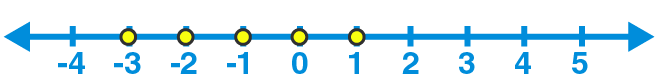10. Solve (x / 3) + (1 / 4) < (x / 6) + (1 / 2), x ε W. Also represent its solution on the number line.

Solution:

Given

(x / 3) + (1 / 4) < (x / 6) + (1 / 2)

(x / 3) – (x / 6) < (1 / 2) – (1 / 4)

(2x – x) / 6 < (2 – 1) / 4

x / 6 < 1 / 4

x < 6 / 4

We get,

x < 3 / 2

As x ε W,

Hence, solution set = {0, 1}

Graphical representation of this solution set is as follows:11. Solve the following inequations and graph their solutions on a number line

(i) – 4 ≤ 4x < 14, x ε N

(ii) – 1 < (x / 2) + 1 ≤ 3, x ε l

Solution:

(i) Given

– 4 ≤ 4x < 14

Dividing by 4, we get,

(-4 / 4) ≤ (4x / 4) < (14 / 4)

-1 ≤ x < 7 / 2

As x ε N,

Therefore, solution set = {1, 2, 3}

The graphical representation for this solution set is as follows:(ii) Given

– 1 < (x / 2) + 1 ≤ 3

By subtracting – 1, we get,

-1 – 1 < {(x / 2) + 1} – 1 ≤ 3 – 1

– 2 < (x / 2) ≤ – 2

Multiplying by 2, we get,

– 2 × 2 < (x / 2) × 2 ≤ – 2 × 2

– 4 < x ≤ – 4

As x ε l,

Therefore, solution set = {-3, -2, -1, 0, 1, 2, 3, 4}

The graphical representation for this solution set is as follows: